# 一，红黑树

## （1）如何定义一棵“红黑树”？

• 根节点是黑色的；
• 每个叶子节点都是黑色的空节点，也就是说，叶子节点不存储数据；
• 任何相邻的节点都不能同时为红色，也就是说，红色节点是被黑色节点隔开的；
• 每个节点，从该节点到达其可达叶子节点的所有路径，都包含相同数目的黑色节点；

## （3）我们刚刚提到了很多平衡二叉查找树，现在我们就来看下，为什么在工程中大家都喜欢用红黑树这种平衡二叉查找树？

AVL 树是一种高度平衡的二叉树，所以查找的效率非常高，但是，有利就有弊，AVL 树为了维持这种高度的平衡，就要付出更多的代价。每次插入、删除都要做调整，就比较复杂、耗时。所以，对于有频繁的插入、删除操作的数据集合，使用 AVL 树的代价就有点高了。

• 红黑树是一种平衡二叉查找树。它是为了解决普通二叉查找树在数据更新的过程中，复杂度退化的问题而产生的。
• 红黑树的高度近似 log2n，所以它是近似平衡，插入、删除、查找操作的时间复杂度都是 O(logn)。
• 因为红黑树是一种性能非常稳定的二叉查找树，所以，在工程中，但凡是用到动态插入、删除、查找数据的场景，都可以用到它。不过，它实现起来比较复杂，如果自己写代码实现，难度会有些高，这个时候，我们其实更倾向用跳表来替代它。

## （4）动态数据结构支持动态地数据插入、删除、查找操作，除了红黑树，我们前面还学习过哪些呢？能对比一下各自的优势、劣势，以及应用场景吗？

• 散列表：插入删除查找都是O(1), 是最常用的，但其缺点是不能顺序遍历以及扩容缩容的性能损耗。适用于那些不需要顺序遍历，数据更新不那么频繁的。
• 跳表：插入删除查找都是O(logn), 并且能顺序遍历。缺点是空间复杂度O(n)。适用于不那么在意内存空间的，其顺序遍历和区间查找非常方便。
• 红黑树：插入删除查找都是O(logn), 中序遍历即是顺序遍历，稳定。缺点是难以实现，去查找不方便。其实跳表更佳，但红黑树已经用于很多地方了。

## （5）左旋和右旋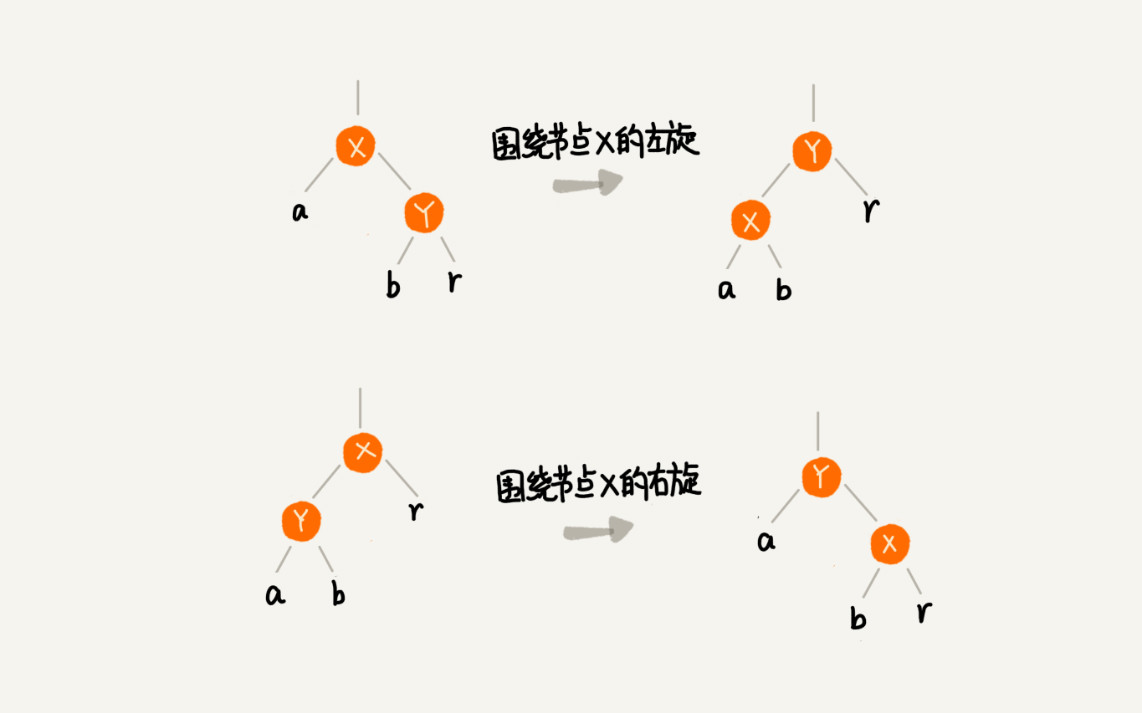# 二，递归树

## 1.分析归并排序复杂度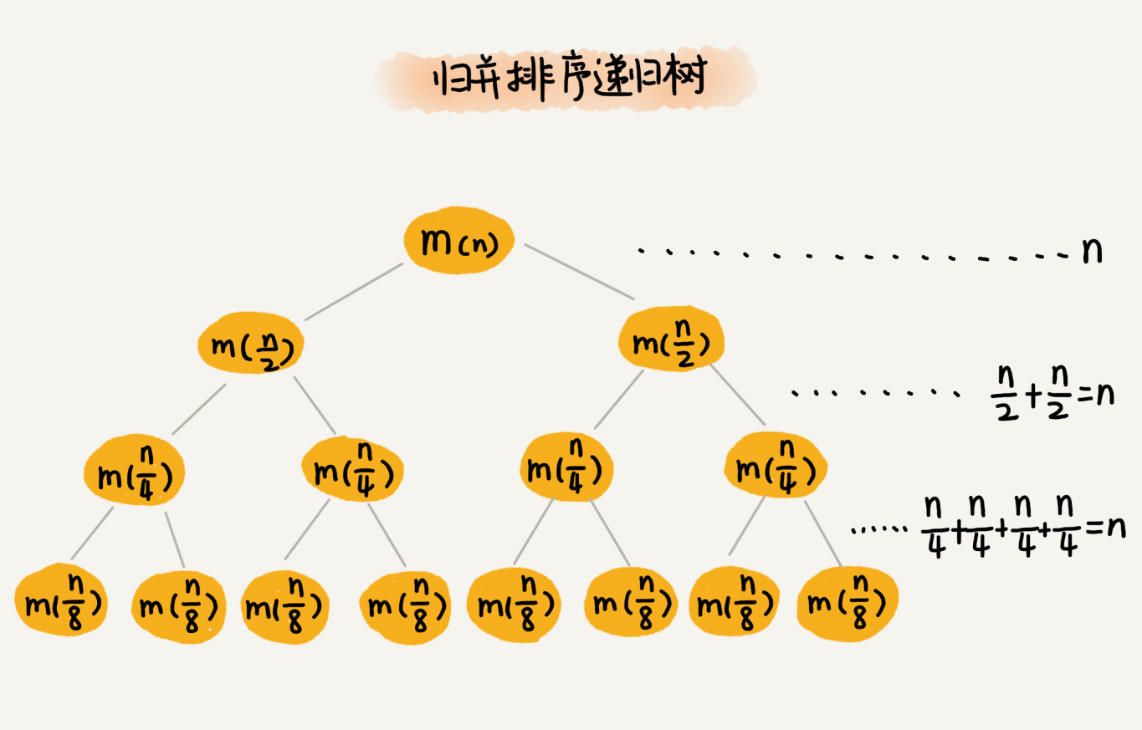## 2.分析快速排序的时间复杂度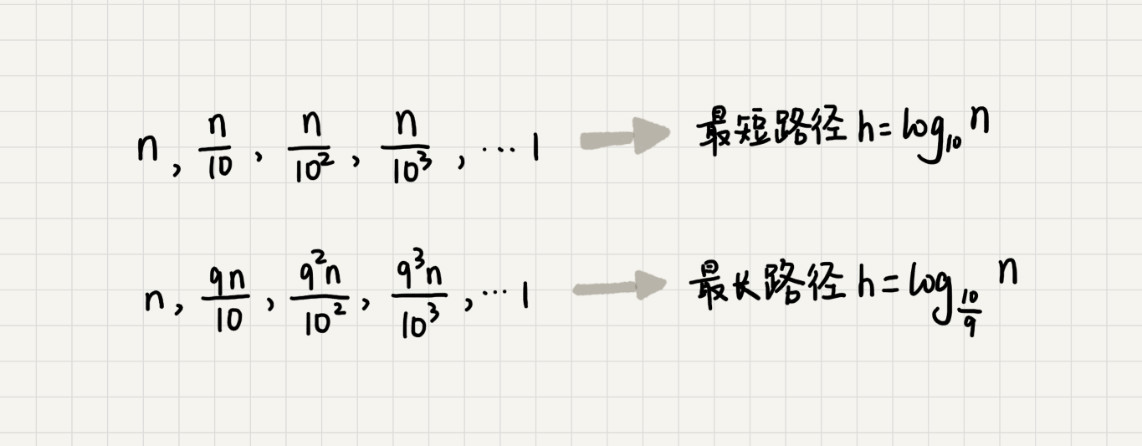## 3.分析斐波那契数列的时间复杂度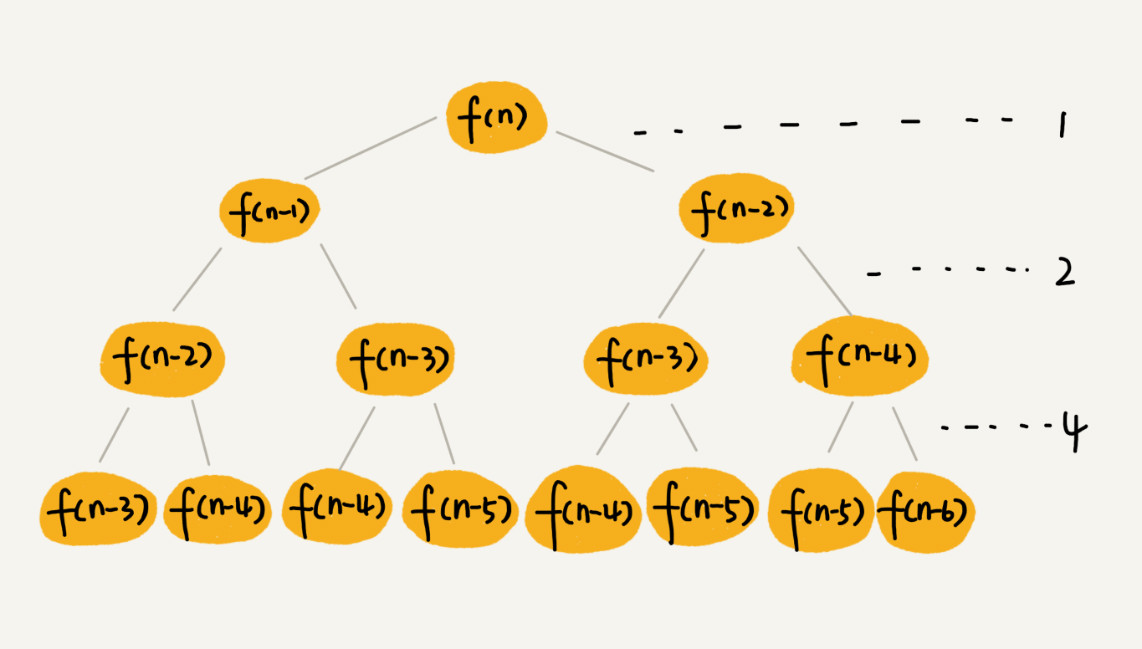f(n) 分解为 f(n−1) 和 f(n−2)，每次数据规模都是 −1 或者 −2，叶子节点的数据规模是 1 或者 2。所以，从根节点走到叶子节点，每条路径是长短不一的。如果每次都是 −1，那最长路径大约就是 n；如果每次都是 −2，那最短路径大约就是 n/2​。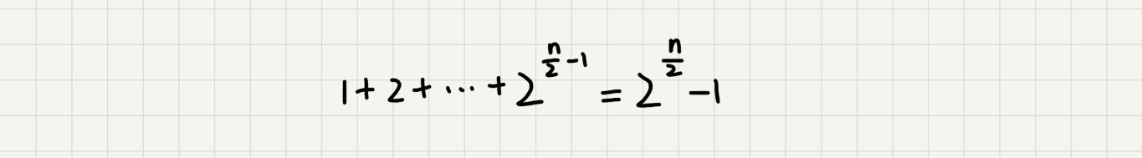## 4.分析全排列的时间复杂度



f(1,2,...n) = {最后一位是1, f(n-1)} + {最后一位是2, f(n-1)} +...+{最后一位是n, f(n-1)}。


// 调用方式：
// int[]a = a={1, 2, 3, 4}; printPermutations(a, 4, 4);
// k表示要处理的子数组的数据个数
public void printPermutations(int[] data, int n, int k) {
if (k == 1) {
for (int i = 0; i < n; ++i) {
System.out.print(data[i] + " ");
}
System.out.println();
}

for (int i = 0; i < k; ++i) {
int tmp = data[i];
data[i] = data[k-1];
data[k-1] = tmp;

printPermutations(data, n, k - 1);

tmp = data[i];
data[i] = data[k-1];
data[k-1] = tmp;
}
}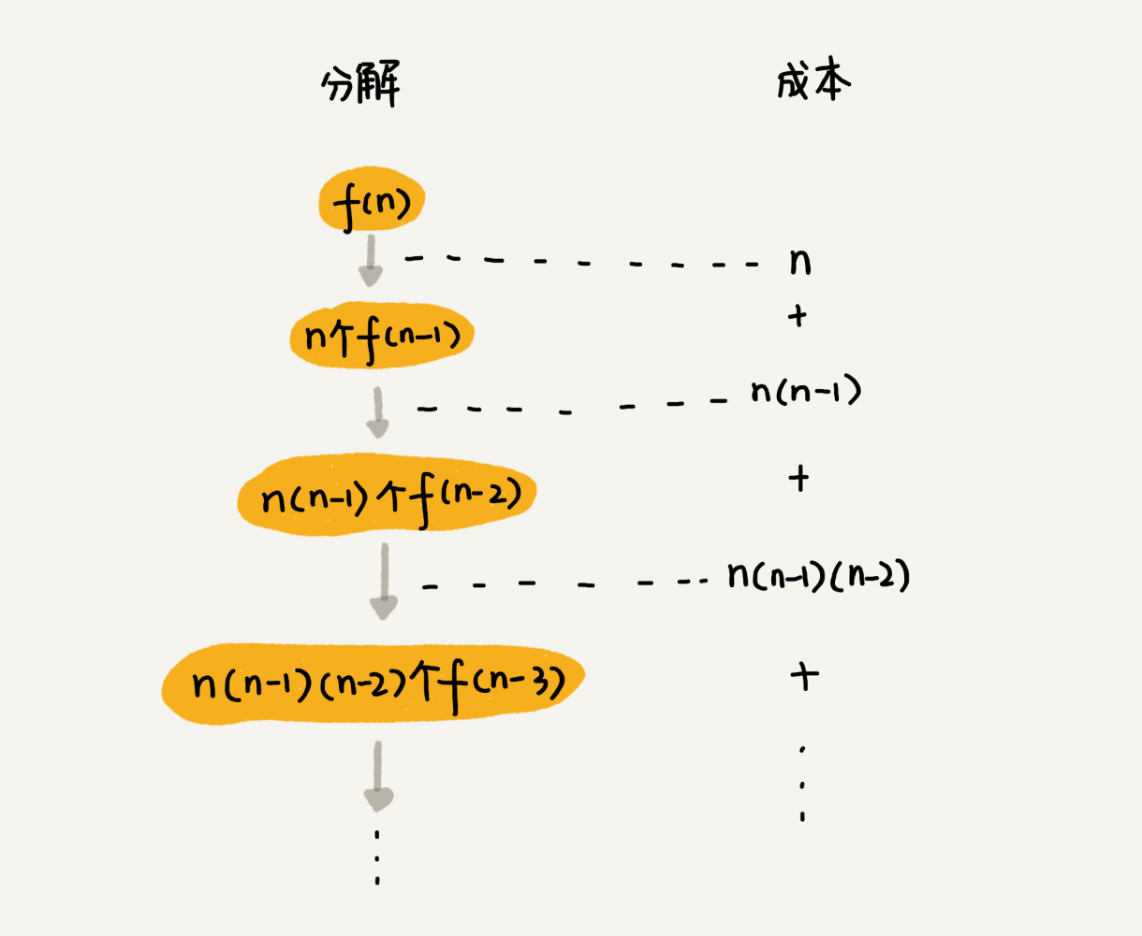n + n*(n-1) + n*(n-1)*(n-2) +... + n*(n-1)*(n-2)*...*2*1

# 三，堆和堆排序

## 1，如何理解“堆”？

• 堆必须是一个完全二叉树
• 堆中的每个节点的值必须大于等于（或者小于等于）其子树中每个节点的值

## 2，往堆中插入一个元素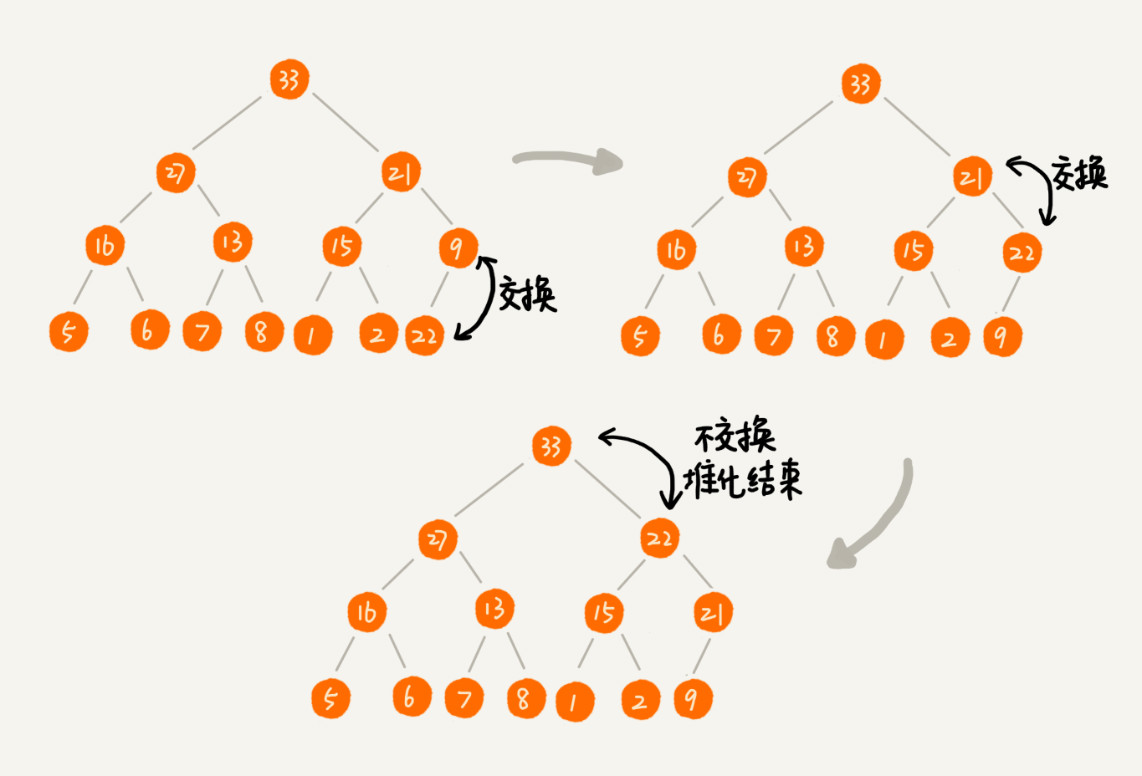public class Heap {
private int[] a; // 数组，从下标1开始存储数据
private int n;  // 堆可以存储的最大数据个数
private int count; // 堆中已经存储的数据个数

public Heap(int capacity) {
a = new int[capacity + 1];
n = capacity;
count = 0;
}

public void insert(int data) {
if (count >= n) return; // 堆满了
++count;
a[count] = data;
int i = count;
while (i/2 > 0 && a[i] > a[i/2]) { // 自下往上堆化
swap(a, i, i/2); // swap()函数作用：交换下标为i和i/2的两个元素
i = i/2;
}
}
}

## 3，删除堆顶元素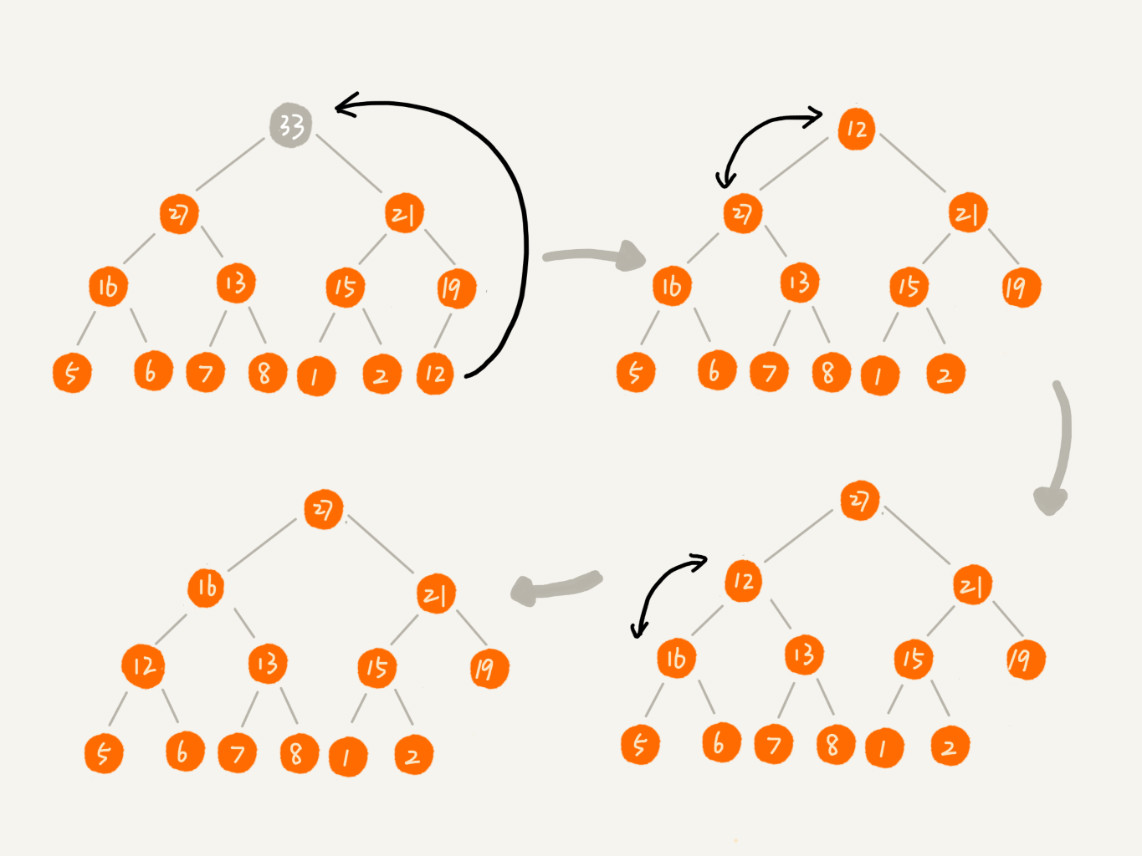public void removeMax() {
if (count == 0) return -1; // 堆中没有数据
a = a[count];
--count;
heapify(a, count, 1);
}

private void heapify(int[] a, int n, int i) { // 自上往下堆化
while (true) {
int maxPos = i;
if (i*2 <= n && a[i] < a[i*2]) maxPos = i*2;
if (i*2+1 <= n && a[maxPos] < a[i*2+1]) maxPos = i*2+1;
if (maxPos == i) break;
swap(a, i, maxPos);
i = maxPos;
}
}

## 4，堆排序实现

### （1）建堆

private static void buildHeap(int[] a, int n) {
for (int i = n/2; i >= 1; --i) {
heapify(a, n, i);
}
}

private static void heapify(int[] a, int n, int i) {
while (true) {
int maxPos = i;
if (i*2 <= n && a[i] < a[i*2]) maxPos = i*2;
if (i*2+1 <= n && a[maxPos] < a[i*2+1]) maxPos = i*2+1;
if (maxPos == i) break;
swap(a, i, maxPos);
i = maxPos;
}
}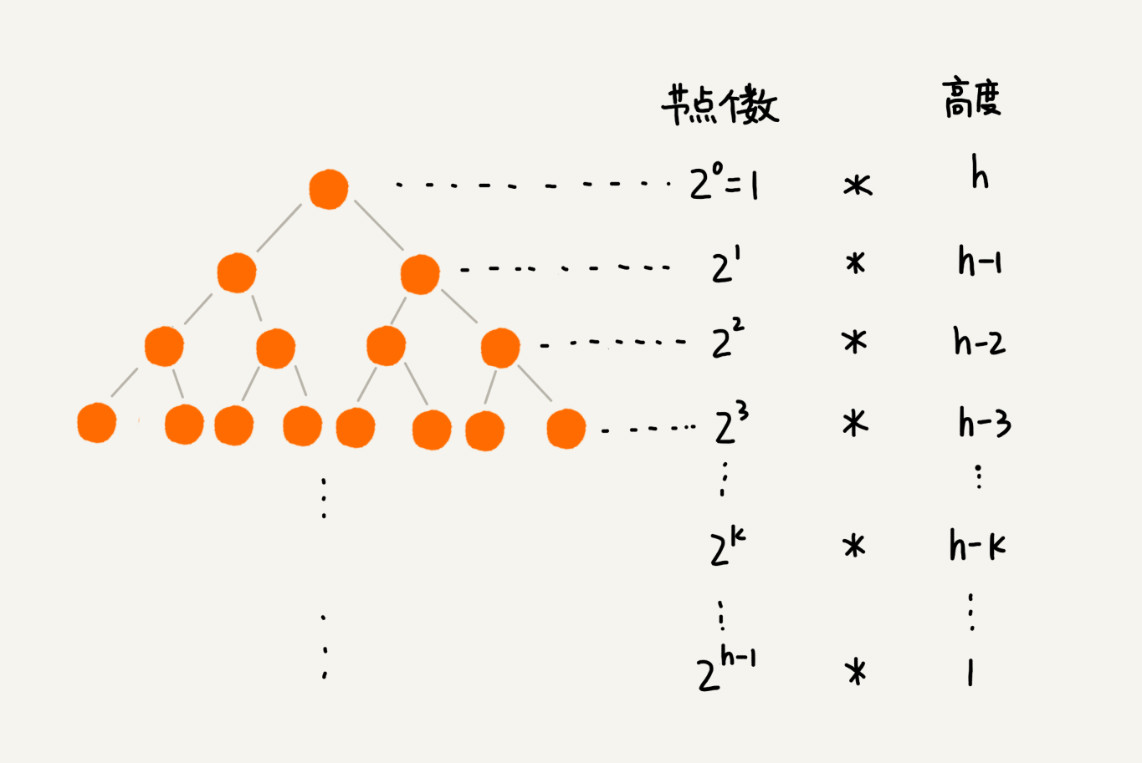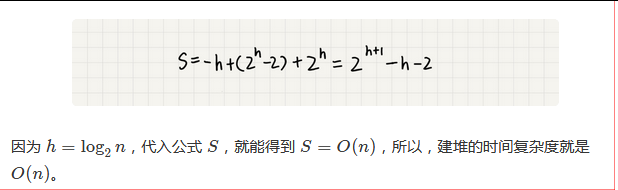### （2）排序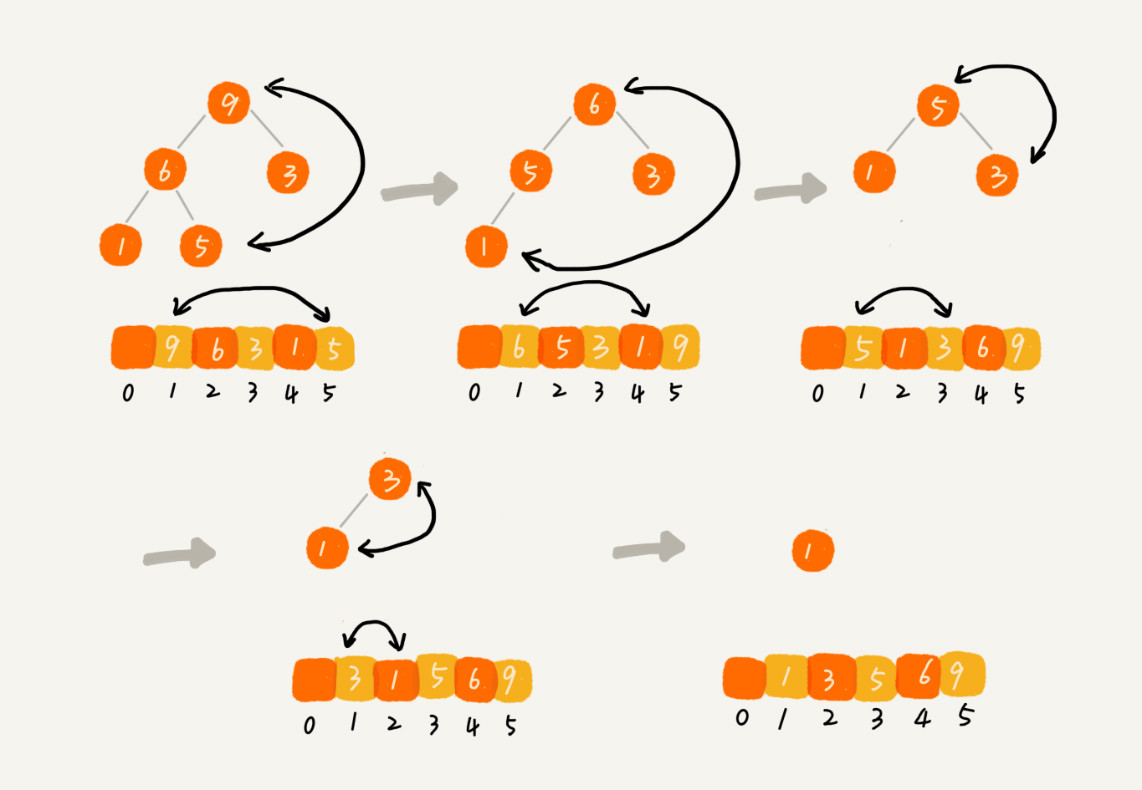// n表示数据的个数，数组a中的数据从下标1到n的位置。
public static void sort(int[] a, int n) {
buildHeap(a, n);
int k = n;
while (k > 1) {
swap(a, 1, k);
--k;
heapify(a, k, 1);
}
}

## 5，为什么快速排序要比堆排序性能好

• 第一点，堆排序数据访问的方式没有快速排序友好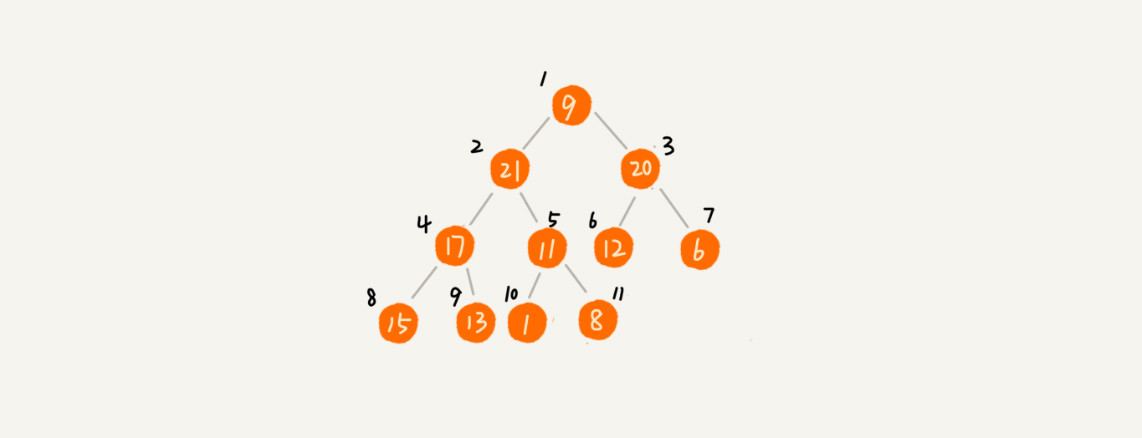• 第二点，对于同样的数据，在排序过程中，堆排序算法的数据交换次数要多于快速排序。

## 6，总结

• 堆是一种完全二叉树。它最大的特性是：每个节点的值都大于等于（或小于等于）其子树节点的值。因此，堆被分成了两类，大顶堆和小顶堆。
• 堆中比较重要的两个操作是插入一个数据和删除堆顶元素。这两个操作都要用到堆化。插入一个数据的时候，我们把新插入的数据放到数组的最后，然后从下往上堆化；删除堆顶数据的时候，我们把数组中的最后一个元素放到堆顶，然后从上往下堆化。这两个操作时间复杂度都是 O(logn)。
• 除此之外，我们还讲了堆的一个经典应用，堆排序。堆排序包含两个过程，建堆和排序。我们将下标从 n/2 到 1 的节点，依次进行从上到下的堆化操作，然后就可以将数组中的数据组织成堆这种数据结构。接下来，我们迭代地将堆顶的元素放到堆的末尾，并将堆的大小减一，然后再堆化，重复这个过程，直到堆中只剩下一个元素，整个数组中的数据就都有序排列了。

# 四，堆的应用

## 3，堆的应用三：利用堆求中位数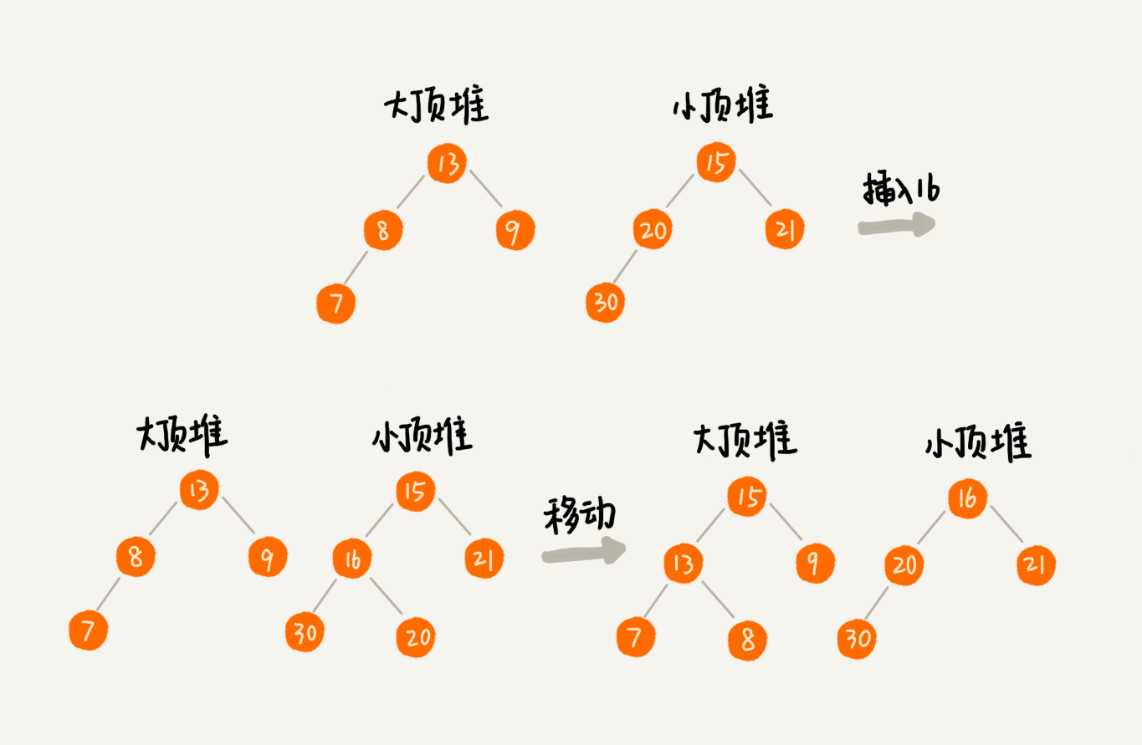## 4，假设现在我们有一个包含 10 亿个搜索关键词的日志文件，如何快速获取到 Top 10 最热门的搜索关键词呢？

### （2）将数据分散存储到不同的文件中

10 亿的关键词还是很多的。我们假设 10 亿条搜索关键词中不重复的有 1 亿条，如果每个搜索关键词的平均长度是 50 个字节，那存储 1 亿个关键词起码需要 5GB 的内存空间，而散列表因为要避免频繁冲突，不会选择太大的装载因子，所以消耗的内存空间就更多了。而我们的机器只有 1GB 的可用内存空间，所以我们无法一次性将所有的搜索关键词加入到内存中。这个时候该怎么办呢？我们在哈希算法那一节讲过，相同数据经过哈希算法得到的哈希值是一样的。我们可以根据哈希算法的这个特点，将 10 亿条搜索关键词先通过哈希算法分片到 10 个文件中。具体可以这样做：我们创建 10 个空文件 00，01，02，……，09。我们遍历这 10 亿个关键词，并且通过某个哈希算法对其求哈希值，然后哈希值同 10 取模，得到的结果就是这个搜索关键词应该被分到的文件编号。

## 5，有一个访问量非常大的新闻网站，我们希望将点击量排名 Top 10 的新闻摘要，滚动显示在网站首页 banner 上，并且每隔 1 小时更新一次。如果你是负责开发这个功能的工程师，你会如何来实现呢？

• 对每篇新闻摘要计算一个hashcode，并建立摘要与hashcode的关联关系，使用map存储，以hashCode为key，新闻摘要为值
• 按每小时一个文件的方式记录下被点击的摘要的hashCode
• 当一个小时结果后，上一个小时的文件被关闭，开始计算上一个小时的点击top10
• 将hashcode分片到多个文件中，通过对hashCode取模运算，即可将相同的hashCode分片到相同的文件中
• 针对每个文件取top10的hashCode，使用Map<hashCode,int>的方式，统计出所有的摘要点击次数，然后再使用小顶堆（大小为10）计算top10
• 再针对所有分片计算一个总的top10,最后合并的逻辑也是使用小顶堆，计算top10
• 如果仅展示前一个小时的top10,计算结束
• 如果需要展示全天，需要与上一次的计算按hashCode进行合并，然后在这合并的数据中取top10
• 在展示时，将计算得到的top10的hashcode，转化为新闻摘要显示即可

## 6，总结

1. 优先级队列是一种特殊的队列，优先级高的数据先出队，而不再像普通的队列那样，先进先出。实际上，堆就可以看作优先级队列，只是称谓不一样罢了。
2. 求 Top K 问题又可以分为针对静态数据和针对动态数据，只需要利用一个堆，就可以做到非常高效率的查询 Top K 的数据。
3. 求中位数实际上还有很多变形，比如求 99 百分位数据、90 百分位数据等，处理的思路都是一样的，即利用两个堆，一个大顶堆，一个小顶堆，随着数据的动态添加，动态调整两个堆中的数据，最后大顶堆的堆顶元素就是要求的数据。

# 五，图

## 1，图的表示

• 判断用户 A 是否关注了用户 B；
• 判断用户 A 是否是用户 B 的粉丝；
• 用户 A 关注用户 B；
• 用户 A 取消关注用户 B；
• 根据用户名称的首字母排序，分页获取用户的粉丝列表；
• 根据用户名称的首字母排序，分页获取用户的关注列表。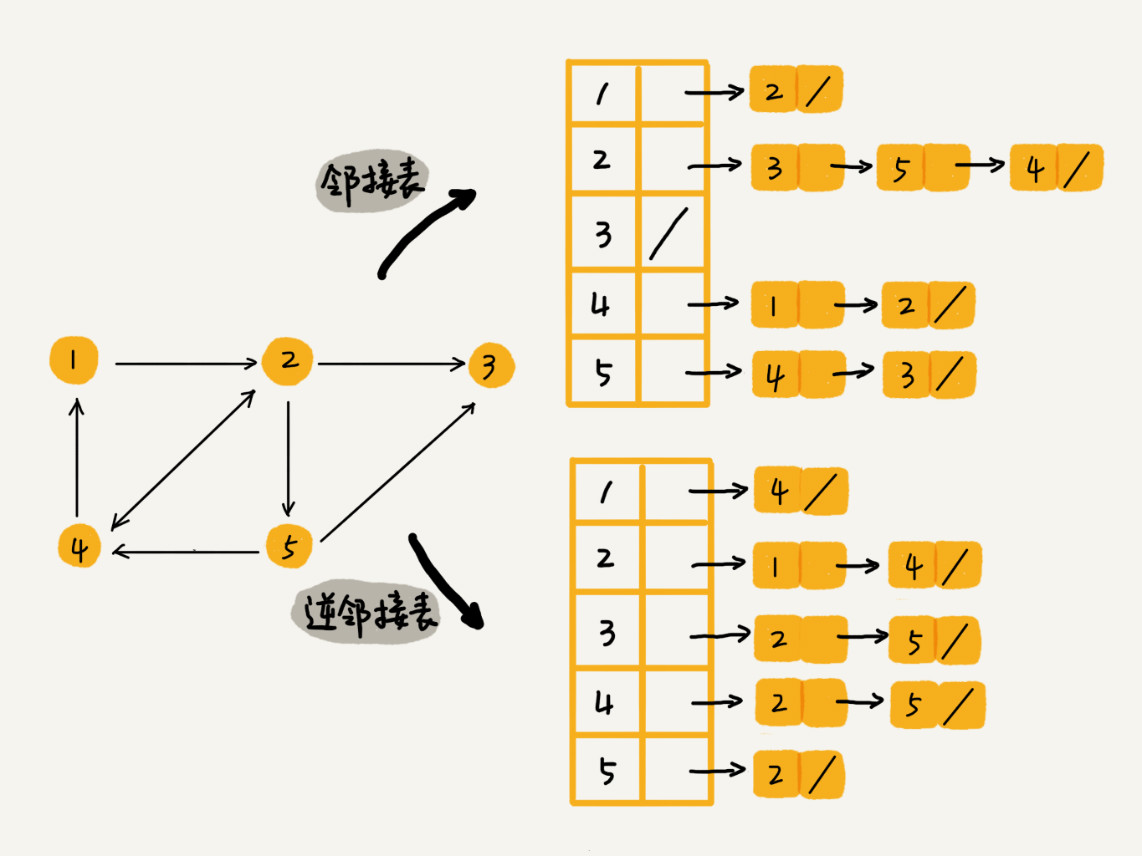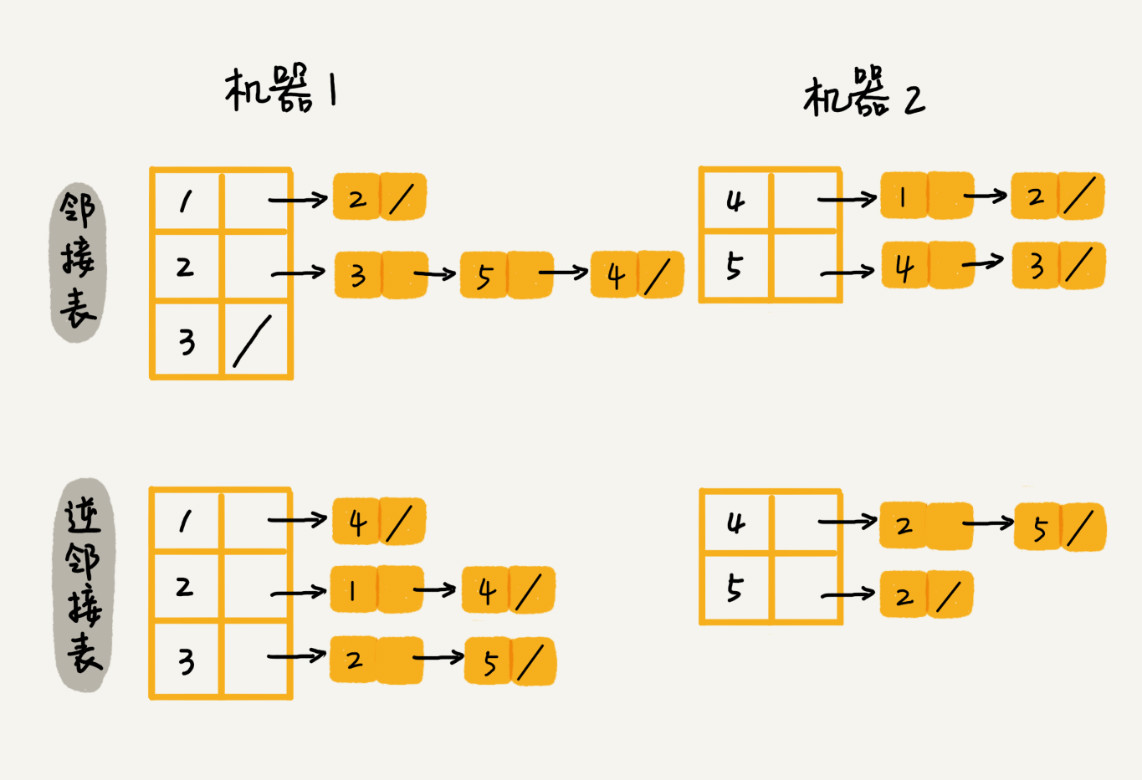## 2，深度和广度优先搜索

public class Graph { // 无向图
private int v; // 顶点的个数

public Graph(int v) {
this.v = v;
for (int i=0; i<v; ++i) {
}
}

public void addEdge(int s, int t) { // 无向图一条边存两次
}
}

### （1）广度优先搜索（BFS）

public void bfs(int s, int t) {
if (s == t) return;
boolean[] visited = new boolean[v];
visited[s]=true;
int[] prev = new int[v];
for (int i = 0; i < v; ++i) {
prev[i] = -1;
}
while (queue.size() != 0) {
int w = queue.poll();
for (int i = 0; i < adj[w].size(); ++i) {
if (!visited[q]) {
prev[q] = w;
if (q == t) {
print(prev, s, t);
return;
}
visited[q] = true;
}
}
}
}

private void print(int[] prev, int s, int t) { // 递归打印s->t的路径
if (prev[t] != -1 && t != s) {
print(prev, s, prev[t]);
}
System.out.print(t + " ");
}

### （2）深度优先搜索（DFS）

public void dfs(int s, int t) {
found = false;
boolean[] visited = new boolean[v];
int[] prev = new int[v];
for (int i = 0; i < v; ++i) {
prev[i] = -1;
}
recurDfs(s, t, visited, prev);
print(prev, s, t);
}

private void recurDfs(int w, int t, boolean[] visited, int[] prev) {
visited[w] = true;
if (w == t) {
return;
}
for (int i = 0; i < adj[w].size(); ++i) {
if (!visited[q]) {
prev[q] = w;
recurDfs(q, t, visited, prev);
}
}
}

# 六，字符串匹配算法

## 1，BF 算法

BF 算法中的 BF 是 Brute Force 的缩写，中文叫作暴力匹配算法，也叫朴素匹配算法。

• 第一，实际的软件开发中，大部分情况下，模式串和主串的长度都不会太长。而且每次模式串与主串中的子串匹配的时候，当中途遇到不能匹配的字符的时候，就可以就停止了，不需要把 m 个字符都比对一下。所以，尽管理论上的最坏情况时间复杂度是 O(n*m)，但是，统计意义上，大部分情况下，算法执行效率要比这个高很多。
• 第二，朴素字符串匹配算法思想简单，代码实现也非常简单。简单意味着不容易出错，如果有 bug 也容易暴露和修复。

## 2，RK 算法

RK 算法的全称叫 Rabin-Karp 算法，是由它的两位发明者 Rabin 和 Karp 的名字来命名的。这个算法理解起来也不是很难。我个人觉得，它其实就是刚刚讲的 BF 算法的升级版。

RK 算法的思路是这样的：我们通过哈希算法对主串中的 n-m+1 个子串分别求哈希值，然后逐个与模式串的哈希值比较大小。如果某个子串的哈希值与模式串相等，那就说明对应的子串和模式串匹配了。因为哈希值是一个数字，数字之间比较是否相等是非常快速的，所以模式串和子串比较的效率就提高了。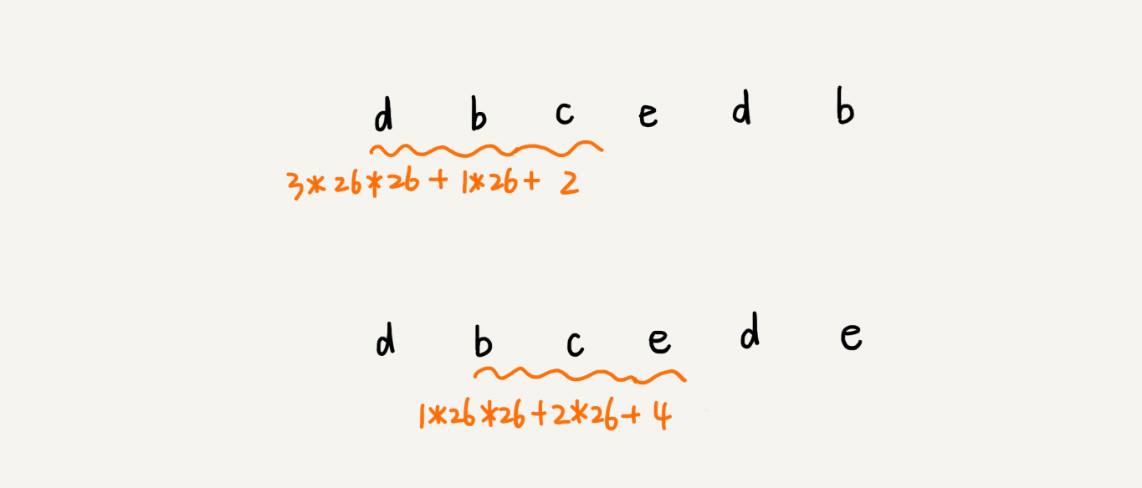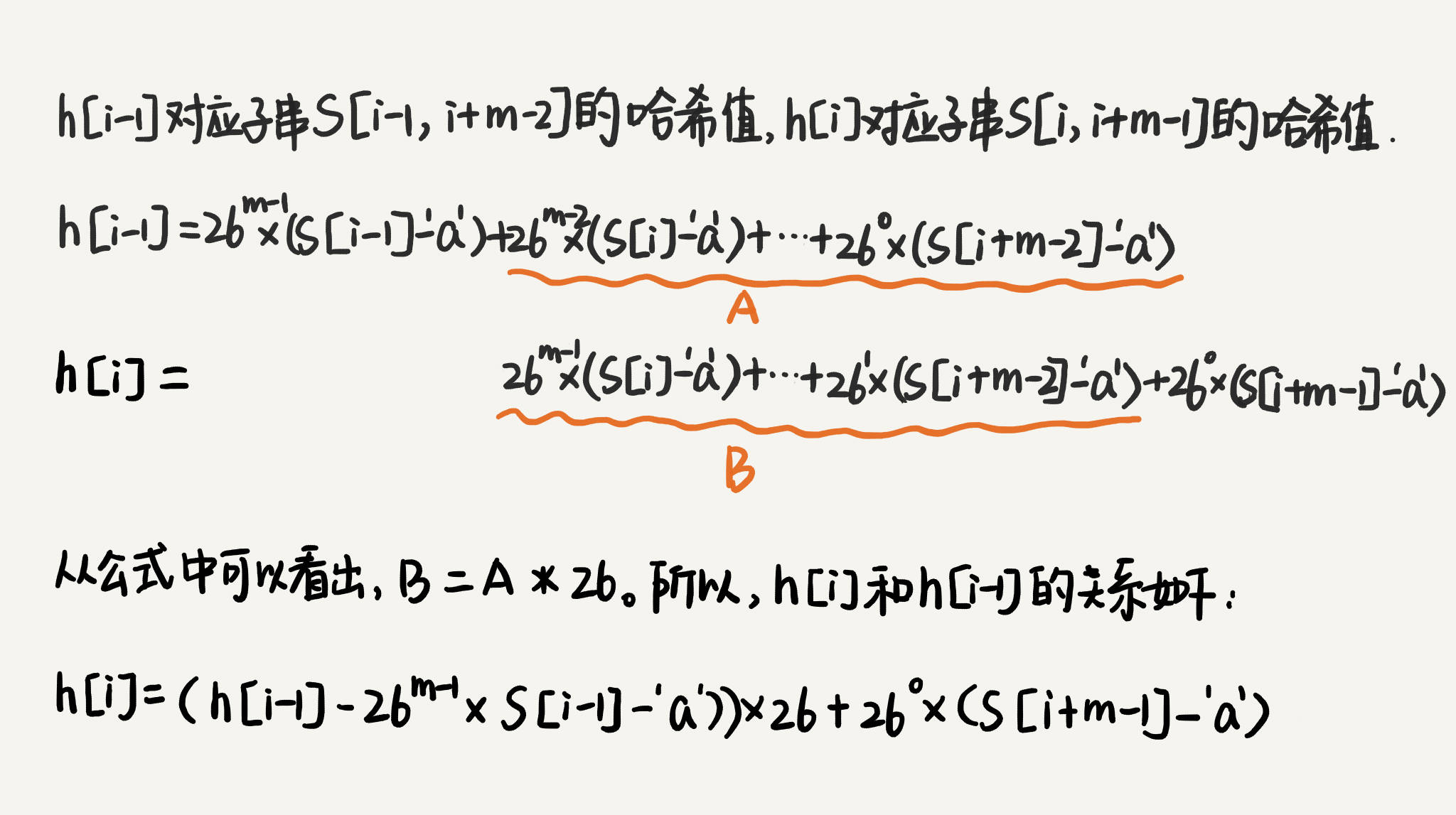## 总结

• BF 算法是最简单、粗暴的字符串匹配算法，它的实现思路是，拿模式串与主串中是所有子串匹配，看是否有能匹配的子串。所以，时间复杂度也比较高，是 O(n*m)，n、m 表示主串和模式串的长度。不过，在实际的软件开发中，因为这种算法实现简单，对于处理小规模的字符串匹配很好用。
• RK 算法是借助哈希算法对 BF 算法进行改造，即对每个子串分别求哈希值，然后拿子串的哈希值与模式串的哈希值比较，减少了比较的时间。所以，理想情况下，RK 算法的时间复杂度是 O(n)，跟 BF 算法相比，效率提高了很多。不过这样的效率取决于哈希算法的设计方法，如果存在冲突的情况下，时间复杂度可能会退化。极端情况下，哈希算法大量冲突，时间复杂度就退化为 O(n*m)。

## 3，BM算法

BM（Boyer-Moore）算法。它是一种非常高效的字符串匹配算法，有实验统计，它的性能是著名的KMP 算法的 3 到 4 倍。

BM 算法核心思想是，利用模式串本身的特点，在模式串中某个字符与主串不能匹配的时候，将模式串往后多滑动几位，以此来减少不必要的字符比较，提高匹配的效率。BM 算法构建的规则有两类，坏字符规则和好后缀规则。好后缀规则可以独立于坏字符规则使用。因为坏字符规则的实现比较耗内存，为了节省内存，我们可以只用好后缀规则来实现 BM 算法。

### （1）坏字符规则

BM 算法的匹配顺序比较特别，它是按照模式串下标从大到小的顺序，倒着匹配的。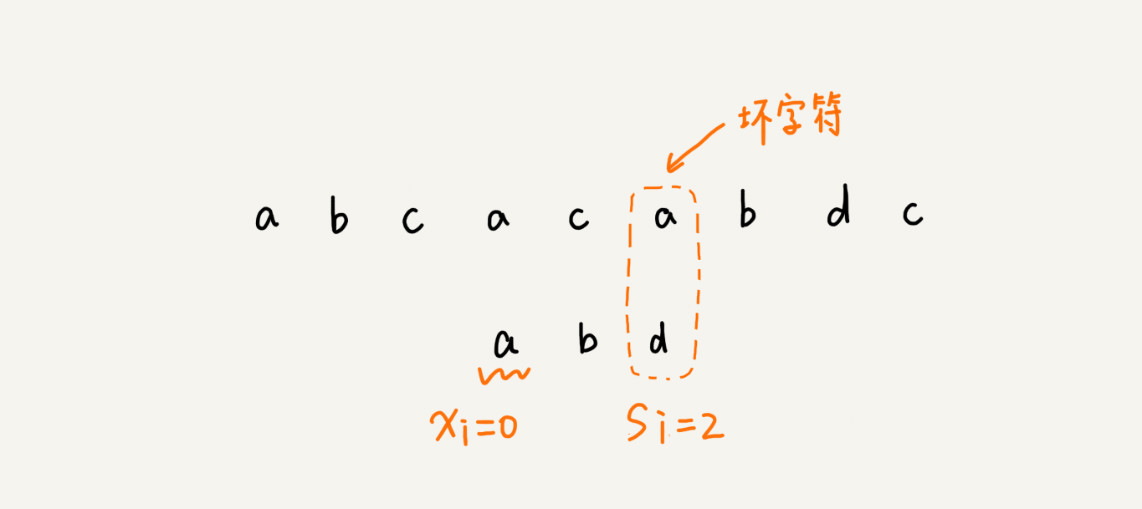private static final int SIZE = 256; // 全局变量或成员变量
private void generateBC(char[] b, int m, int[] bc) {
for (int i = 0; i < SIZE; ++i) {
bc[i] = -1; // 初始化bc
}
for (int i = 0; i < m; ++i) {
int ascii = (int)b[i]; // 计算b[i]的ASCII值
bc[ascii] = i;
}
}

### （2）好后缀规则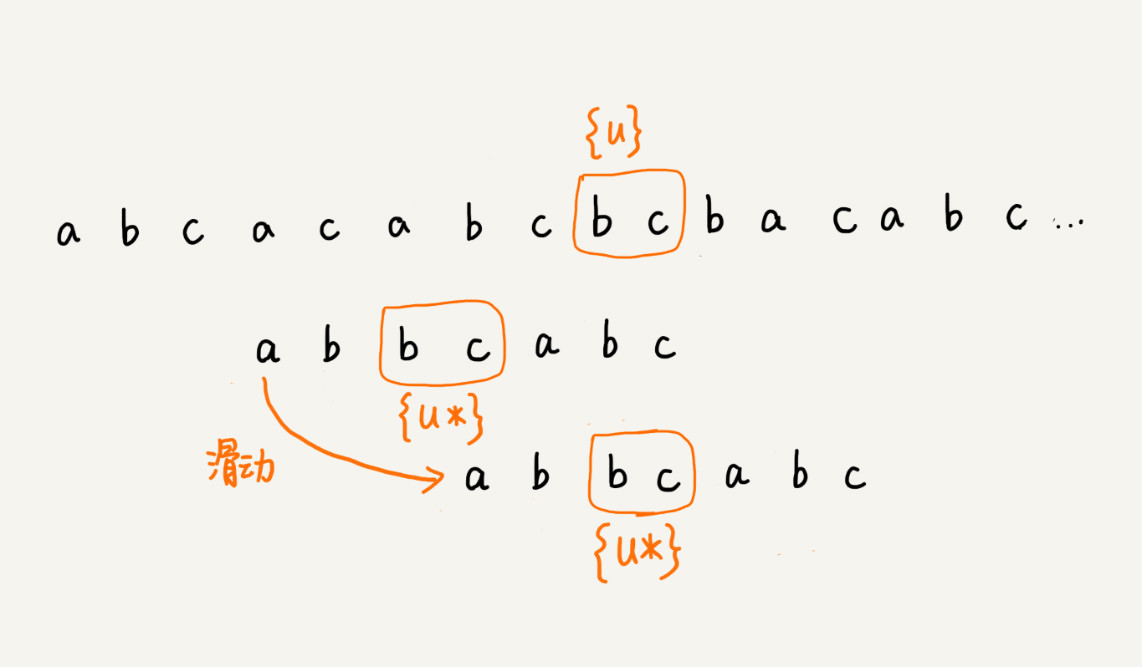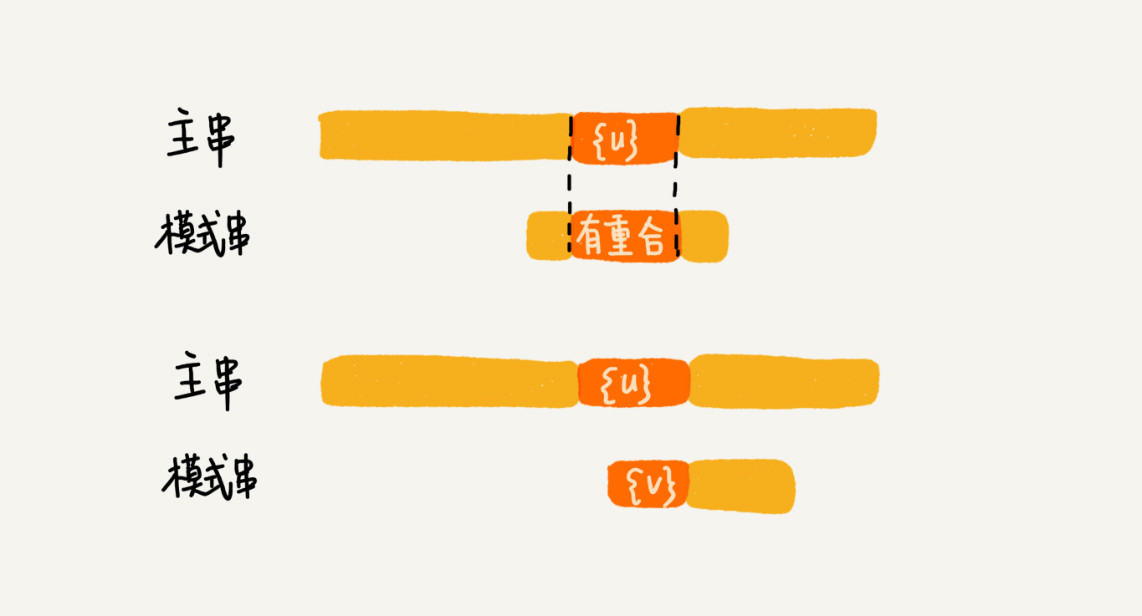## 4，KMP算法

### （1）KMP 算法基本原理

KMP 算法是根据三位作者（D.E.Knuth，J.H.Morris 和 V.R.Pratt）的名字来命名的，算法的全称是 Knuth Morris Pratt 算法，简称为 KMP 算法。KMP 算法的核心思想：

在模式串和主串匹配的过程中，把不能匹配的那个字符仍然叫作坏字符，把已经匹配的那段字符串叫作好前缀。当遇到坏字符的时候，我们就要把模式串往后滑动，在滑动的过程中，只要模式串和好前缀有上下重合，前面几个字符的比较，就相当于拿好前缀的后缀子串，跟模式串的前缀子串在比较。这个比较的过程能否更高效了呢？可以不用一个字符一个字符地比较了吗？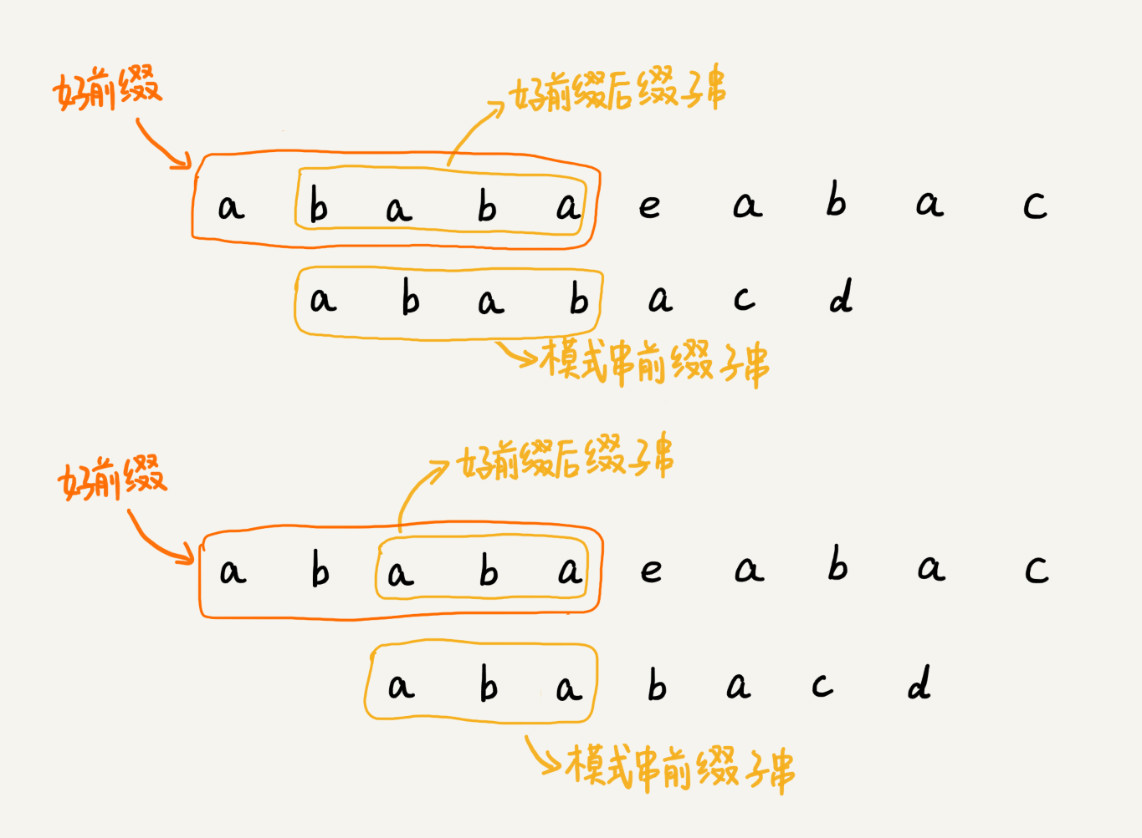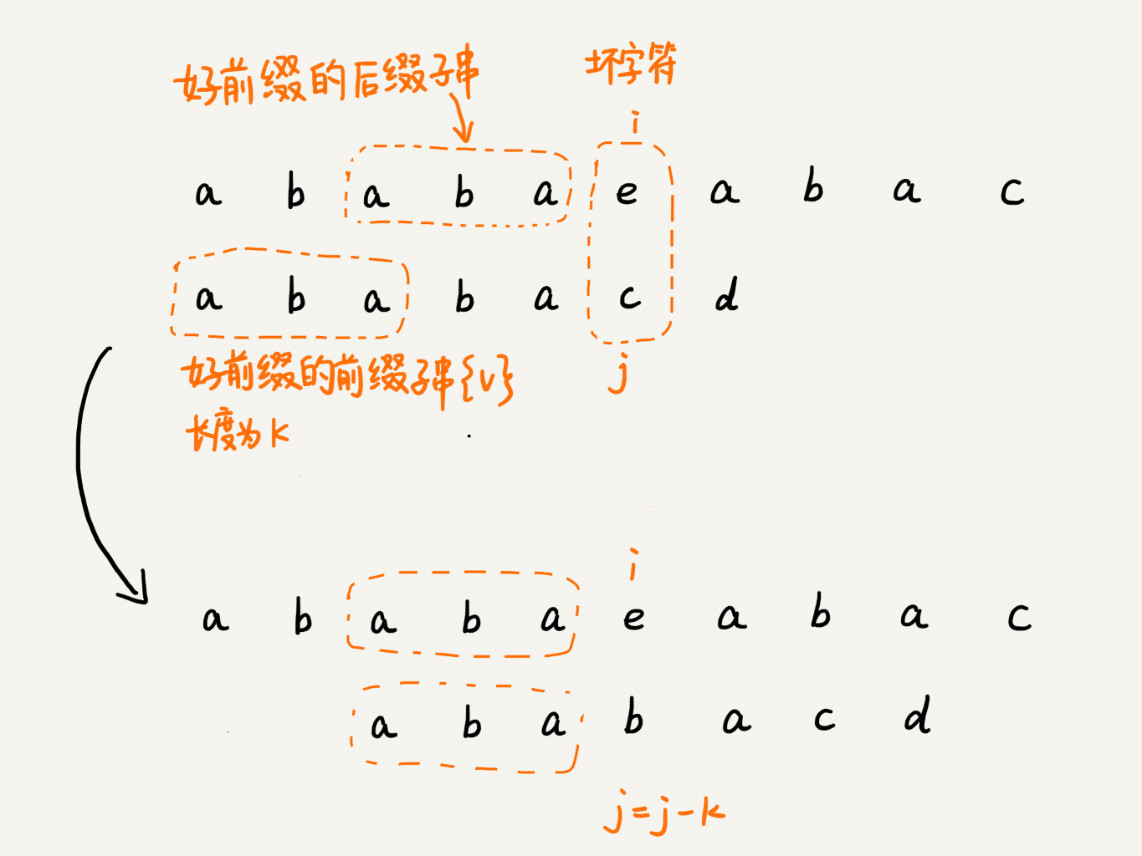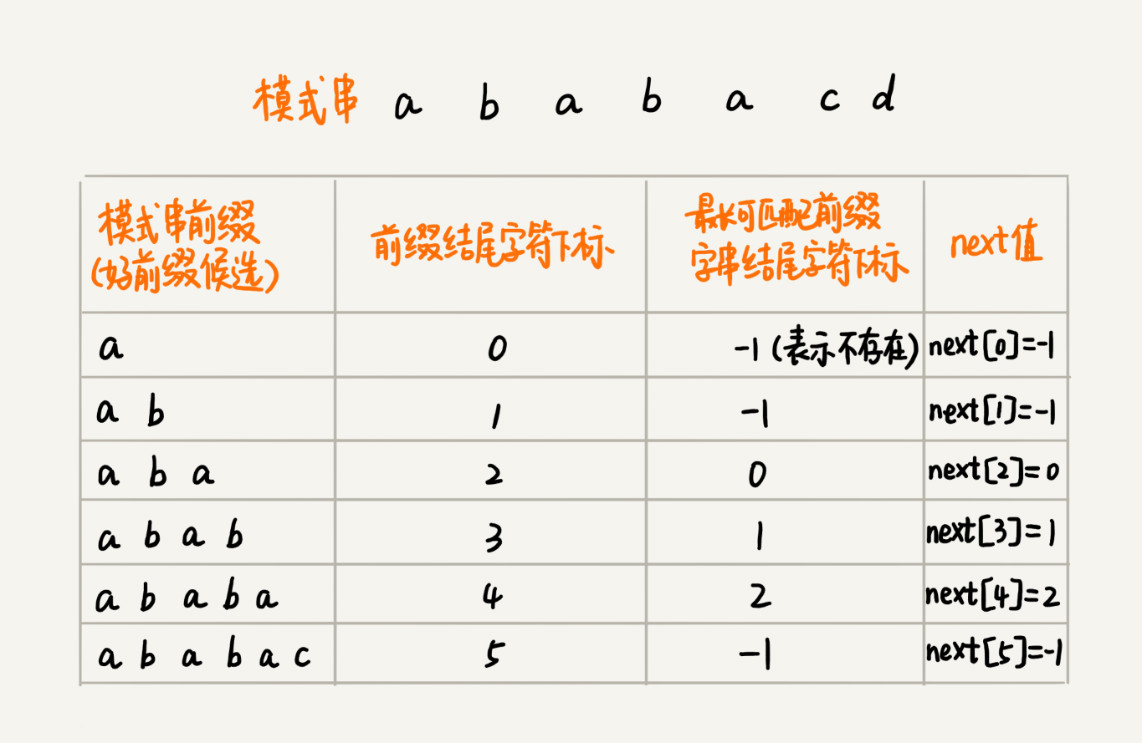// a, b分别是主串和模式串；n, m分别是主串和模式串的长度。
public static int kmp(char[] a, int n, char[] b, int m) {
int[] next = getNexts(b, m);
int j = 0;
for (int i = 0; i < n; ++i) {
while (j > 0 && a[i] != b[j]) { // 如果遇到坏字符，从最长匹配前缀子串开始找，j回退，i不变，一直找到a[i]和b[j]
j = next[j - 1] + 1;
}
if (a[i] == b[j]) {
++j;
}
if (j == m) { // 找到匹配模式串的了
return i - m + 1;
}
}
return -1;
}

### （2）失效函数计算方法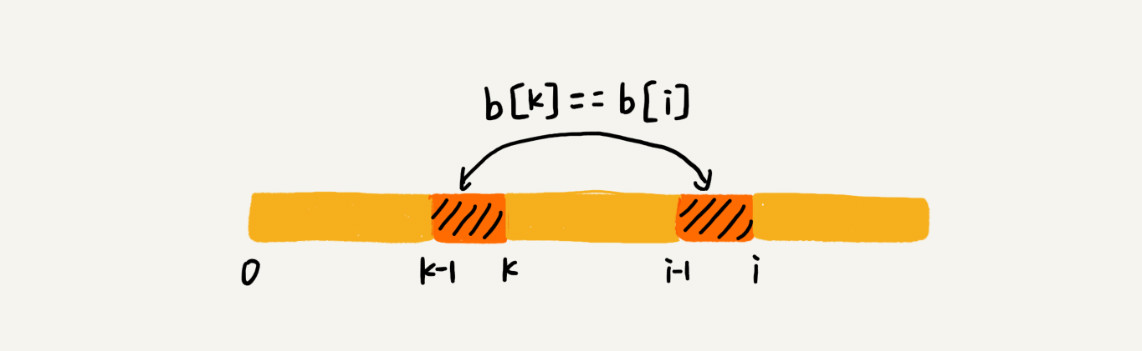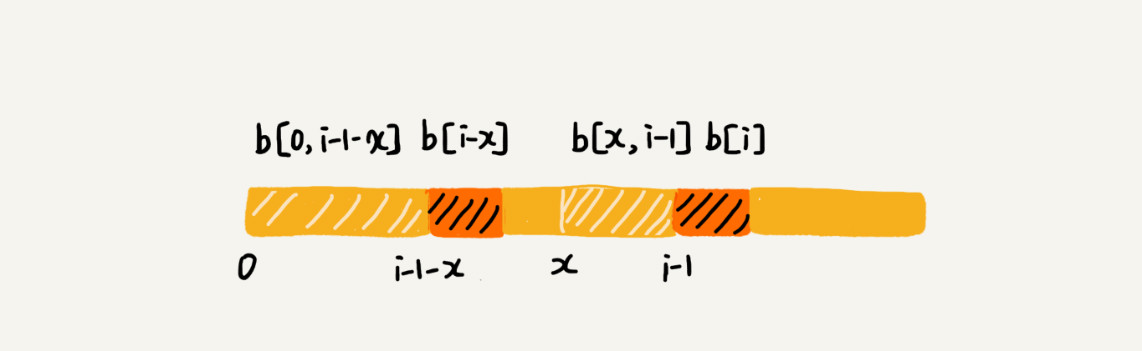// b表示模式串，m表示模式串的长度
private static int[] getNexts(char[] b, int m) {
int[] next = new int[m];
next = -1;
int k = -1;
for (int i = 1; i < m; ++i) {
while (k != -1 && b[k + 1] != b[i]) {
k = next[k];
}
if (b[k + 1] == b[i]) {
++k;
}
next[i] = k;
}
return next;
}

### （3）KMP 算法复杂度分析

KMP 算法包含两部分，第一部分是构建 next 数组，第二部分才是借助 next 数组匹配。所以，关于时间复杂度，我们要分别从这两部分来分析。

• 计算 next 数组的代码中，我们可以找一些参照变量，i 和 k。i 从 1 开始一直增加到 m，而 k 并不是每次 for 循环都会增加，所以，k 累积增加的值肯定小于 m。而 while 循环里 k=next[k]，实际上是在减小 k 的值，k 累积都没有增加超过 m，所以 while 循环里面 k=next[k] 总的执行次数也不可能超过 m。因此，next 数组计算的时间复杂度是 O(m)。
• i 从 0 循环增长到 n-1，j 的增长量不可能超过 i，所以肯定小于 n。而 while 循环中的那条语句 j=next[j-1]+1，不会让 j 增长的，那有没有可能让 j 不变呢？也没有可能。因为 next[j-1] 的值肯定小于 j-1，所以 while 循环中的这条语句实际上也是在让 j 的值减少。而 j 总共增长的量都不会超过 n，那减少的量也不可能超过 n，所以 while 循环中的这条语句总的执行次数也不会超过 n，所以这部分的时间复杂度是 O(n)。

### （4）总结

KMP 算法和上一节讲的 BM 算法的本质非常类似，都是根据规律在遇到坏字符的时候，把模式串往后多滑动几位。BM 算法有两个规则，坏字符和好后缀。KMP 算法借鉴 BM 算法的思想，可以总结成好前缀规则。这里面最难懂的就是 next 数组的计算。如果用最笨的方法来计算，确实不难，但是效率会比较低。所以，我讲了一种类似动态规划的方法，按照下标 i 从小到大，依次计算 next[i]，并且 next[i] 的计算通过前面已经计算出来的 next，next，……，next[i-1] 来推导。

# 七，Trie树

Trie 树，也叫“字典树”。顾名思义，它是一个树形结构。它是一种专门处理字符串匹配的数据结构，用来解决在一组字符串集合中快速查找某个字符串的问题。Trie 树的本质，就是利用字符串之间的公共前缀，将重复的前缀合并在一起。

## 1，如何实现一棵 Trie 树？

Trie 树主要有两个操作，一个是将字符串集合构造成 Trie 树。这个过程分解开来的话，就是一个将字符串插入到 Trie 树的过程。另一个是在 Trie 树中查询一个字符串。

public class Trie {
private TrieNode root = new TrieNode('/'); // 存储无意义字符

// 往Trie树中插入一个字符串
public void insert(char[] text) {
TrieNode p = root;
for (int i = 0; i < text.length; ++i) {
int index = text[i] - 'a';
if (p.children[index] == null) {
TrieNode newNode = new TrieNode(text[i]);
p.children[index] = newNode;
}
p = p.children[index];
}
p.isEndingChar = true;
}

// 在Trie树中查找一个字符串
public boolean find(char[] pattern) {
TrieNode p = root;
for (int i = 0; i < pattern.length; ++i) {
int index = pattern[i] - 'a';
if (p.children[index] == null) {
return false; // 不存在pattern
}
p = p.children[index];
}
if (p.isEndingChar == false) return false; // 不能完全匹配，只是前缀
else return true; // 找到pattern
}

public class TrieNode {
public char data;
public TrieNode[] children = new TrieNode;
public boolean isEndingChar = false;
public TrieNode(char data) {
this.data = data;
}
}
}

## 2，Trie 树很耗内存

Trie 树的本质是避免重复存储一组字符串的相同前缀子串，但是现在每个字符（对应一个节点）的存储远远大于 1 个字节。按照我们上面举的例子，数组长度为 26，每个元素是 8 字节，那每个节点就会额外需要 26*8=208 个字节。而且这还是只包含 26 个字符的情况。如果字符串中不仅包含小写字母，还包含大写字母、数字、甚至是中文，那需要的存储空间就更多了。所以，也就是说，在某些情况下，Trie 树不一定会节省存储空间。在重复的前缀并不多的情况下，Trie 树不但不能节省内存，还有可能会浪费更多的内存。当然，我们不可否认，Trie 树尽管有可能很浪费内存，但是确实非常高效。

## 3，Trie 树与散列表、红黑树的比较

• 第一，字符串中包含的字符集不能太大。我们前面讲到，如果字符集太大，那存储空间可能就会浪费很多。即便可以优化，但也要付出牺牲查询、插入效率的代价。
• 第二，要求字符串的前缀重合比较多，不然空间消耗会变大很多。
• 第三，如果要用 Trie 树解决问题，那我们就要自己从零开始实现一个 Trie 树，还要保证没有 bug，这个在工程上是将简单问题复杂化，除非必须，一般不建议这样做。
• 第四，我们知道，通过指针串起来的数据块是不连续的，而 Trie 树中用到了指针，所以，对缓存并不友好，性能上会打个折扣。

## 4，总结

### 如何利用 Trie 树，实现搜索关键词的提示功能？

Trie 树是一种解决字符串快速匹配问题的数据结构。如果用来构建 Trie 树的这一组字符串中，前缀重复的情况不是很多，那 Trie 树这种数据结构总体上来讲是比较费内存的，是一种空间换时间的解决问题思路。尽管比较耗费内存，但是对内存不敏感或者内存消耗在接受范围内的情况下，在 Trie 树中做字符串匹配还是非常高效的，时间复杂度是 O(k)，k 表示要匹配的字符串的长度。

# 八，AC自动机

## 1，基于单模式串和 Trie 树实现的敏感词过滤

BF 算法、RK 算法、BM 算法、KMP 算法，还有 Trie 树。前面四种算法都是单模式串匹配算法，只有 Trie 树是多模式串匹配算法。单模式串匹配算法，是在一个模式串和一个主串之间进行匹配，也就是说，在一个主串中查找一个模式串。多模式串匹配算法，就是在多个模式串和一个主串之间做匹配，也就是说，在一个主串中查找多个模式串。

## 2，经典的多模式串匹配算法：AC 自动机

AC 自动机算法，全称是 Aho-Corasick 算法。其实，Trie 树跟 AC 自动机之间的关系，就像单串匹配中朴素的串匹配算法，跟 KMP 算法之间的关系一样，只不过前者针对的是多模式串而已。所以，AC 自动机实际上就是在 Trie 树之上，加了类似 KMP 的 next 数组，只不过此处的 next 数组是构建在树上罢了。如果代码表示，就是下面这个样子：

public class AcNode {
public char data;
public AcNode[] children = new AcNode; // 字符集只包含a~z这26个字符
public boolean isEndingChar = false; // 结尾字符为true
public int length = -1; // 当isEndingChar=true时，记录模式串长度
public AcNode fail; // 失败指针
public AcNode(char data) {
this.data = data;
}
}

• 将多个模式串构建成 Trie 树；
• 在 Trie 树上构建失败指针（相当于 KMP 中的失效函数 next 数组）。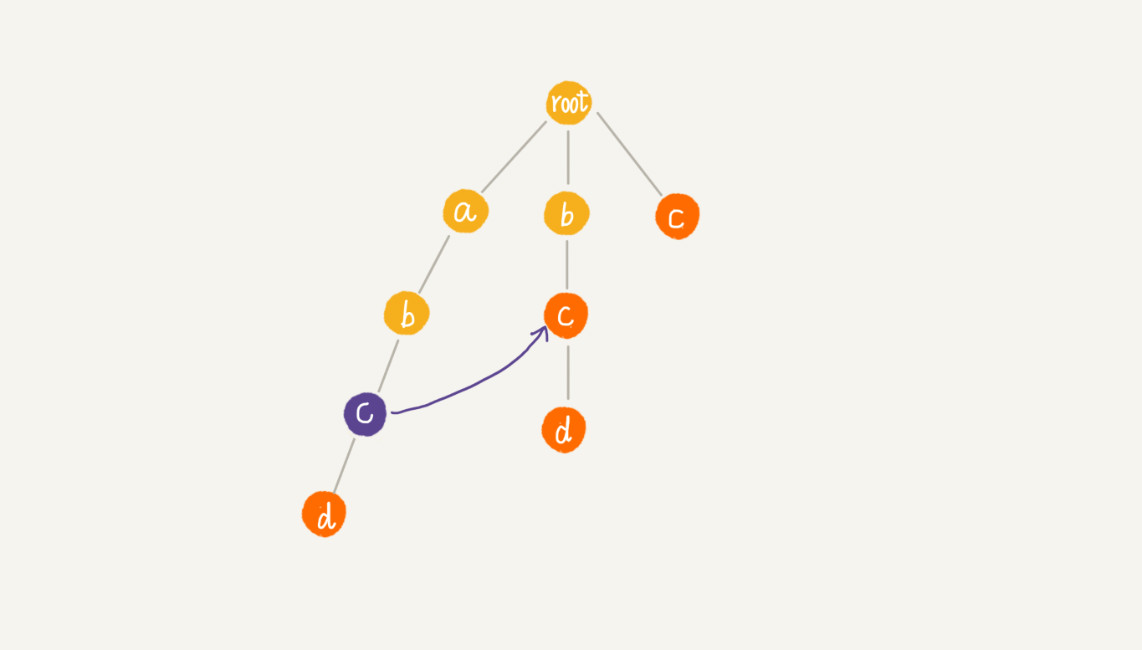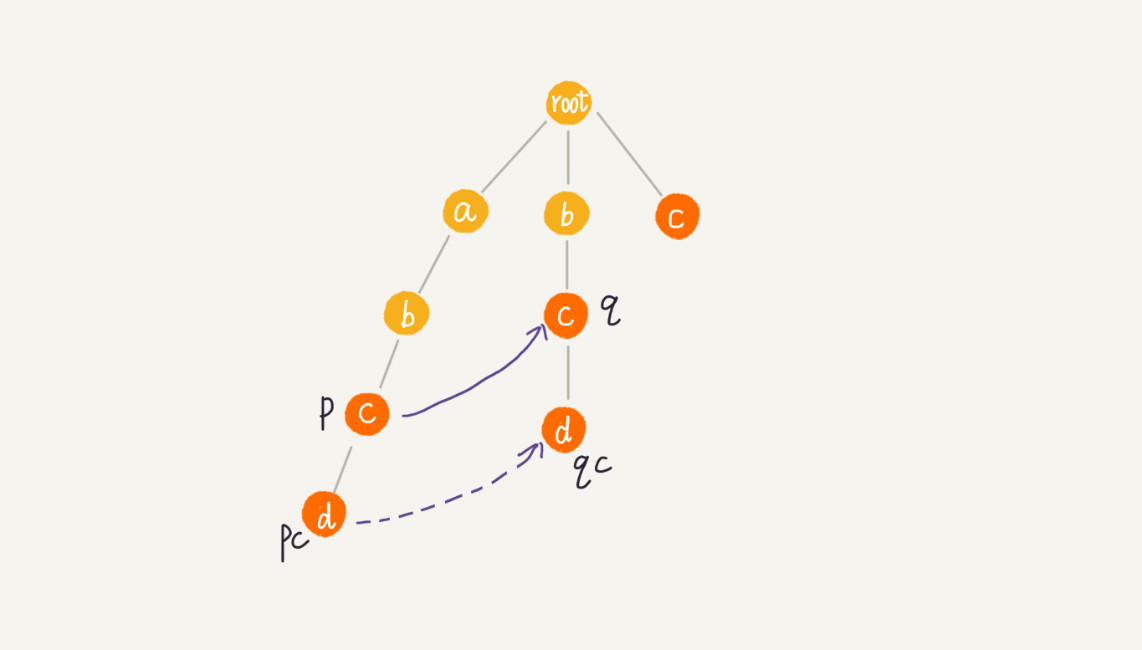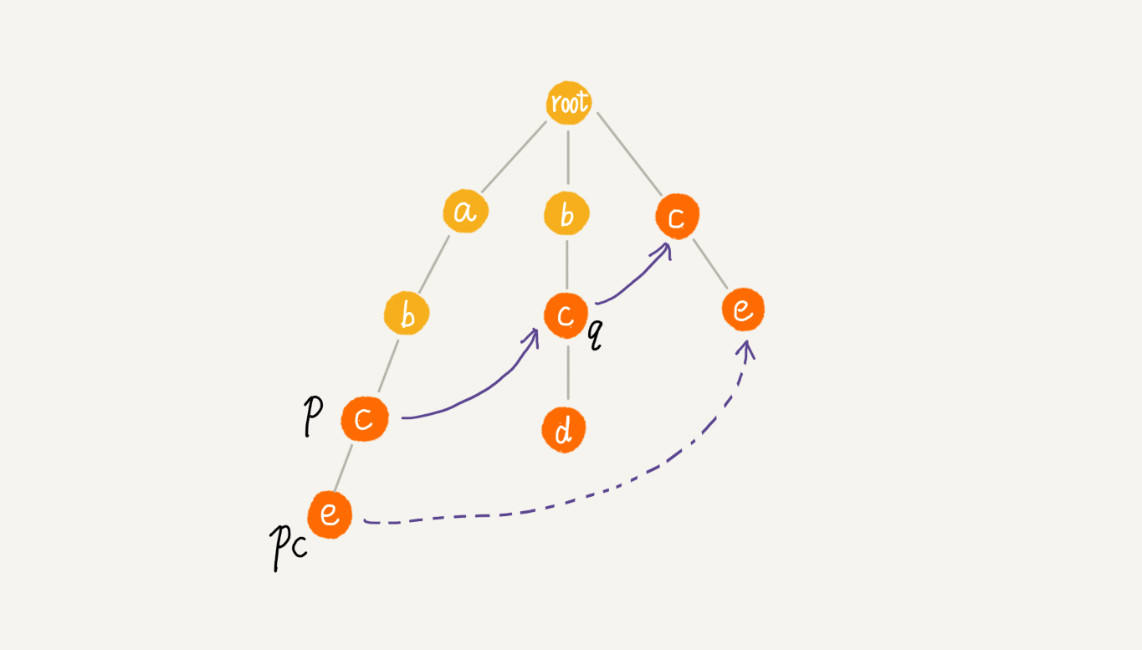public void buildFailurePointer() {
root.fail = null;
while (!queue.isEmpty()) {
AcNode p = queue.remove();
for (int i = 0; i < 26; ++i) {
AcNode pc = p.children[i];
if (pc == null) continue;
if (p == root) {
pc.fail = root;
} else {
AcNode q = p.fail;
while (q != null) {
AcNode qc = q.children[pc.data - 'a'];
if (qc != null) {
pc.fail = qc;
break;
}
q = q.fail;
}
if (q == null) {
pc.fail = root;
}
}
}
}
}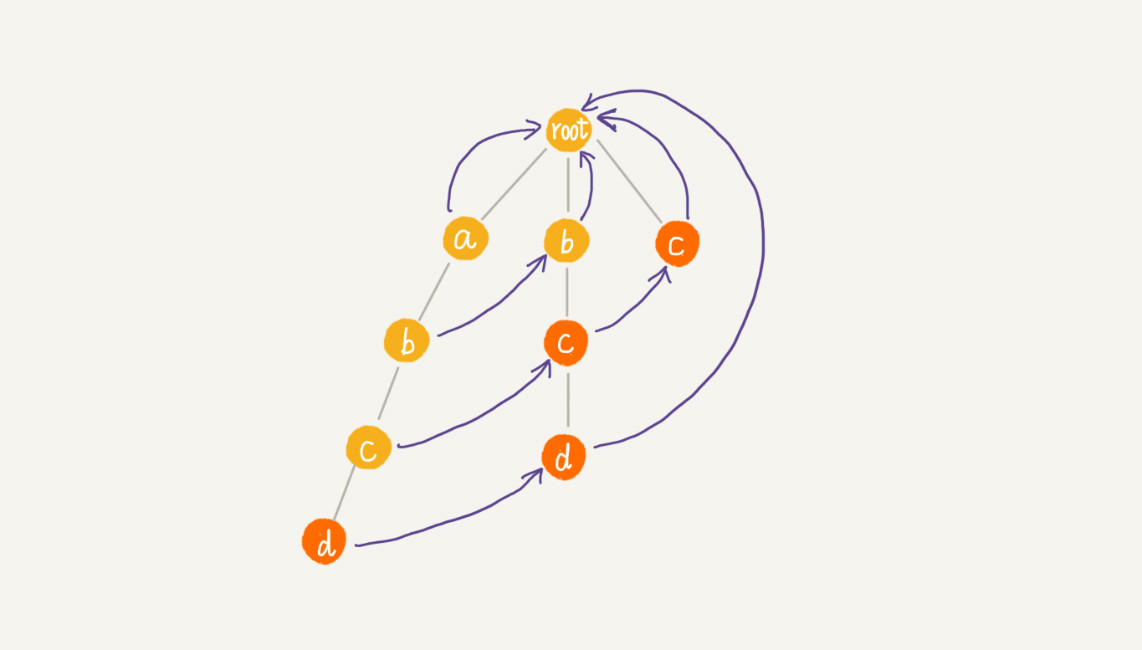AC 自动机到此就构建完成了。我们现在来看下，如何在 AC 自动机上匹配主串？

public void match(char[] text) { // text是主串
int n = text.length;
AcNode p = root;
for (int i = 0; i < n; ++i) {
int idx = text[i] - 'a';
while (p.children[idx] == null && p != root) {
p = p.fail; // 失败指针发挥作用的地方
}
p = p.children[idx];
if (p == null) p = root; // 如果没有匹配的，从root开始重新匹配
AcNode tmp = p;
while (tmp != root) { // 打印出可以匹配的模式串
if (tmp.isEndingChar == true) {
int pos = i-tmp.length+1;
System.out.println("匹配起始下标" + pos + "; 长度" + tmp.length);
}
tmp = tmp.fail;
}
}
}

## 3，AC自动机的时间复杂度

AC 自动机实现的敏感词过滤系统，是否比单模式串匹配方法更高效呢？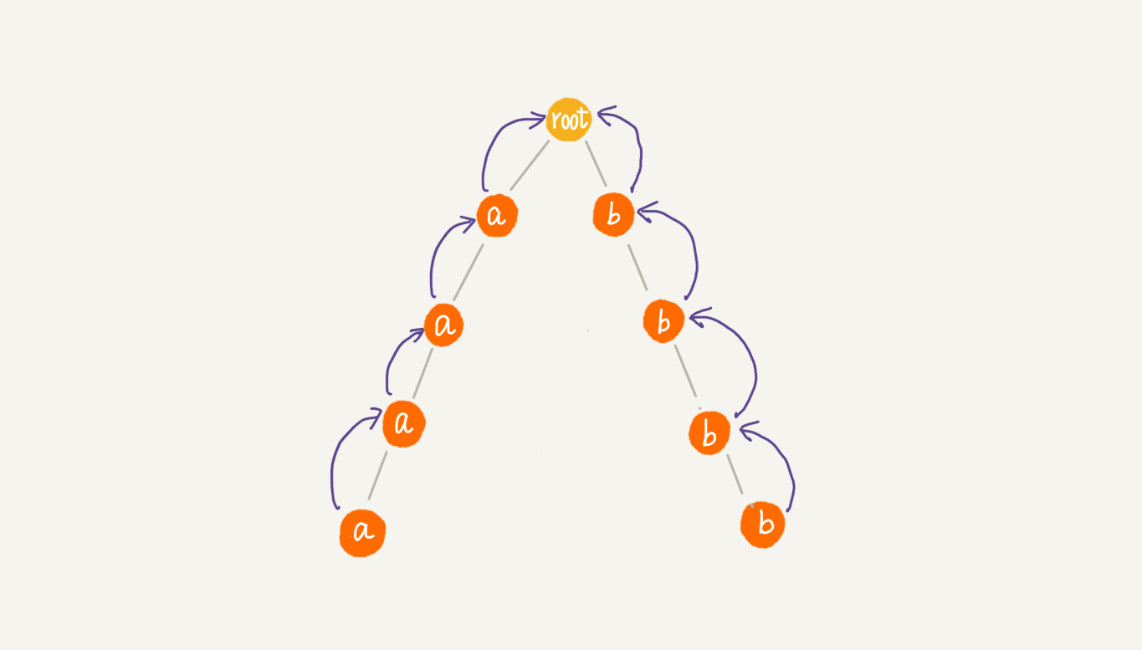# 九，贪心算法

## 1，使用贪心算法的例子

### （2）区间覆盖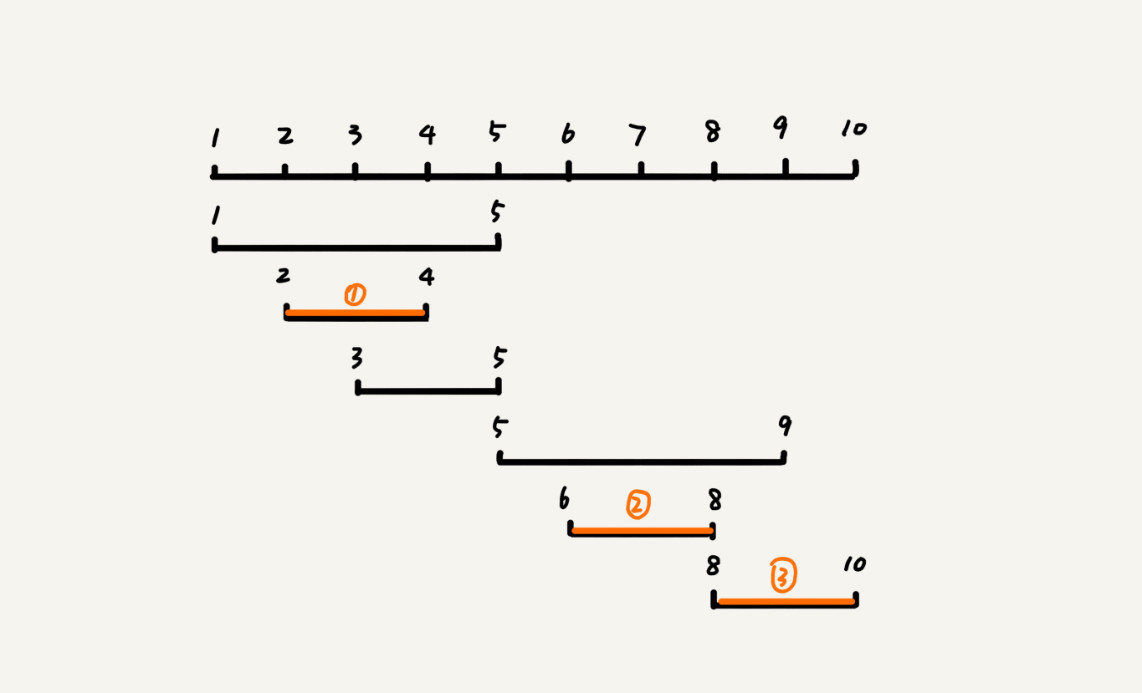### （3）霍夫曼编码

a(000)、b(001)、c(010)、d(011)、e(100)、f(101)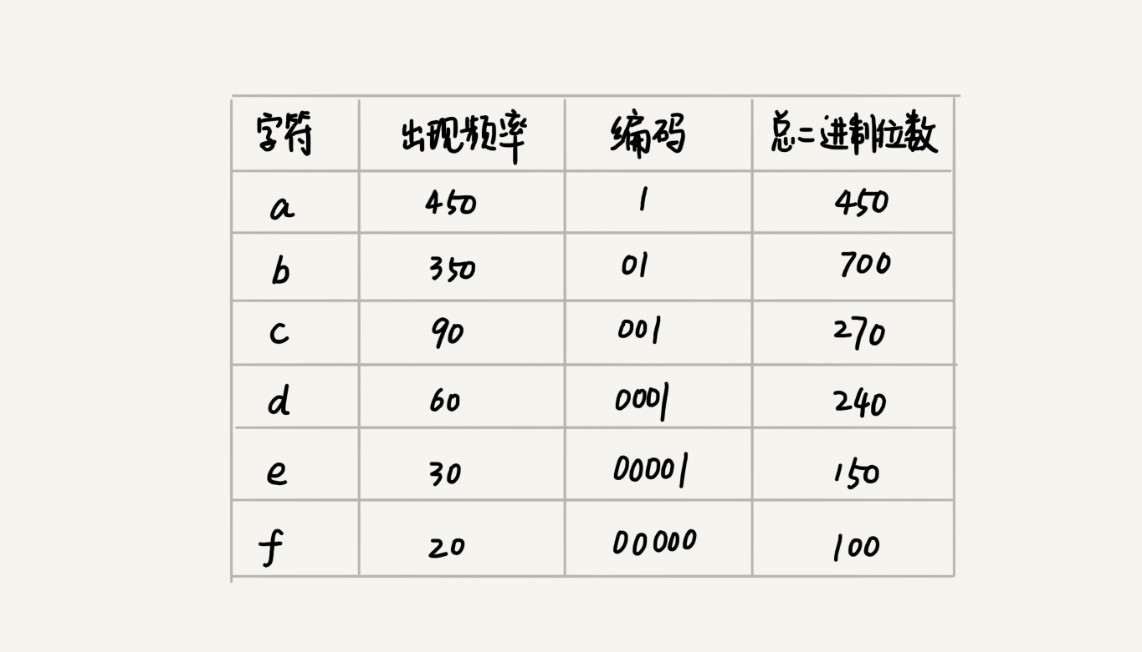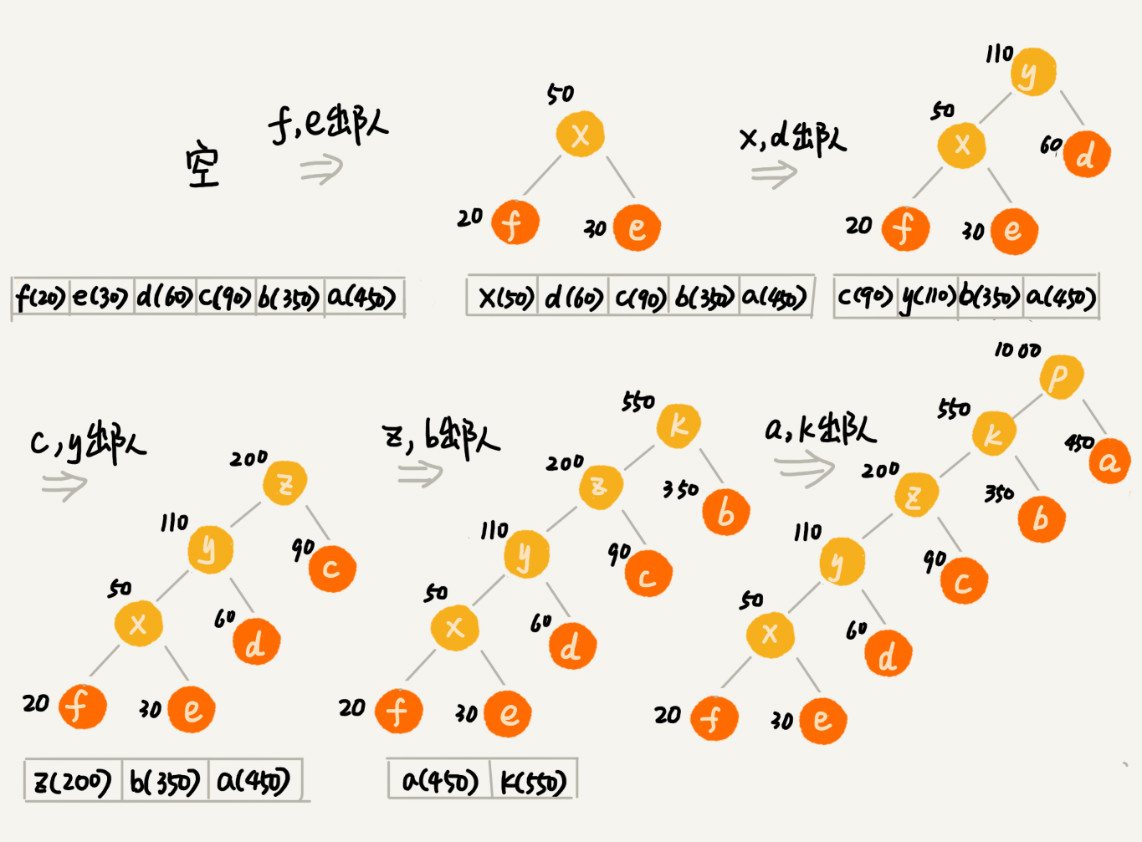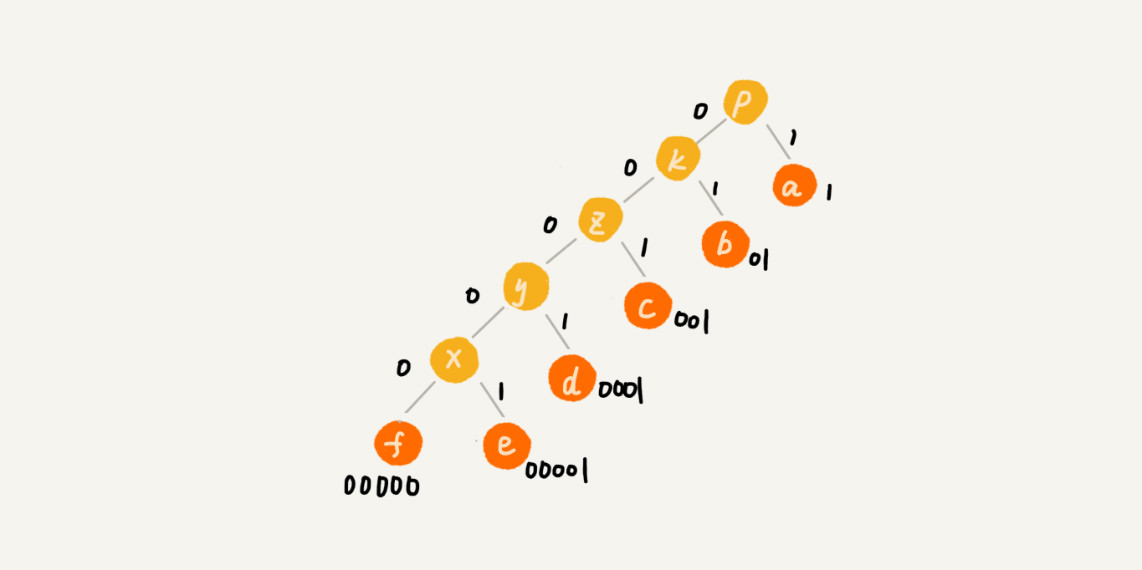## 3，思考题

### 在一个非负整数 a 中，我们希望从中移除 k 个数字，让剩下的数字值最小，如何选择移除哪 k 个数字呢？

4556847594546移除5位：455647594546-->45547594546-->4547594546-->4447594546-->444594546

# 十，分治算法

• 分解：将原问题分解成一系列子问题；
• 解决：递归地求解各个子问题，若子问题足够小，则直接求解；
• 合并：将子问题的结果合并成原问题。

• 原问题与分解成的小问题具有相同的模式；
• 原问题分解成的子问题可以独立求解，子问题之间没有相关性，这一点是分治算法跟动态规划的明显区别，等我们讲到动态规划的时候，会详细对比这两种算法；
• 具有分解终止条件，也就是说，当问题足够小时，可以直接求解；
• 可以将子问题合并成原问题，而这个合并操作的复杂度不能太高，否则就起不到减小算法总体复杂度的效果了。

## 1，分治算法应用举例分析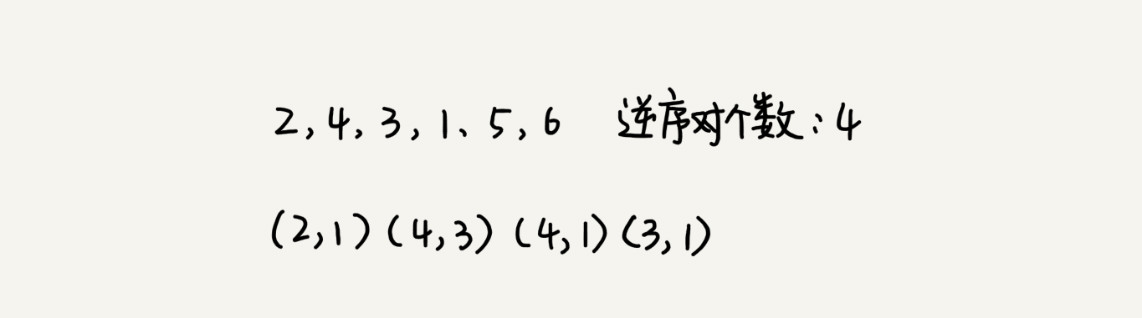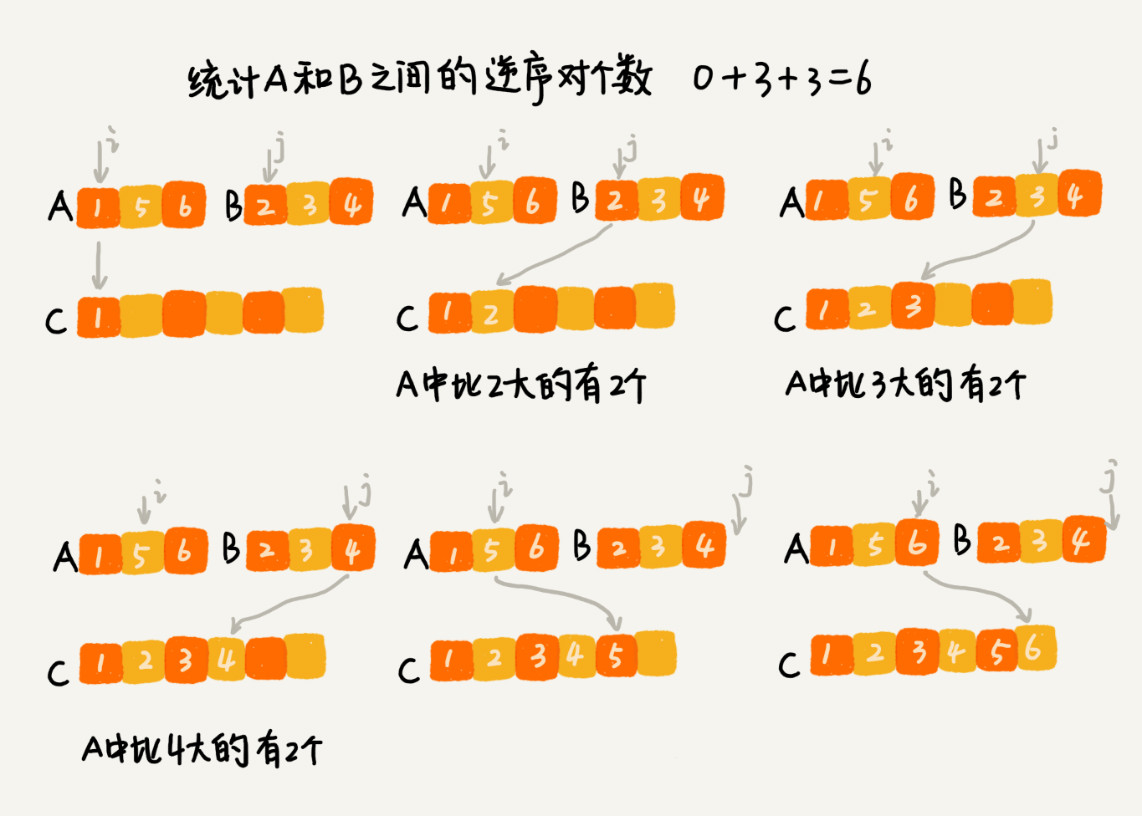private int num = 0; // 全局变量或者成员变量

public int count(int[] a, int n) {
num = 0;
mergeSortCounting(a, 0, n-1);
return num;
}

private void mergeSortCounting(int[] a, int p, int r) {
if (p >= r) return;
int q = (p+r)/2;
mergeSortCounting(a, p, q);
mergeSortCounting(a, q+1, r);
merge(a, p, q, r);
}

private void merge(int[] a, int p, int q, int r) {
int i = p, j = q+1, k = 0;
int[] tmp = new int[r-p+1];
while (i<=q && j<=r) {
if (a[i] <= a[j]) {
tmp[k++] = a[i++];
} else {
num += (q-i+1); // 统计p-q之间，比a[j]大的元素个数
tmp[k++] = a[j++];
}
}
while (i <= q) { // 处理剩下的
tmp[k++] = a[i++];
}
while (j <= r) { // 处理剩下的
tmp[k++] = a[j++];
}
for (i = 0; i <= r-p; ++i) { // 从tmp拷贝回a
a[p+i] = tmp[i];
}
}

# 十一，回溯算法

## 1，八皇后问题


int[] result = new int;//全局或成员变量,下标表示行,值表示queen存储在哪一列
public void cal8queens(int row) { // 调用方式：cal8queens(0);
if (row == 8) { // 8个棋子都放置好了，打印结果
printQueens(result);
return; // 8行棋子都放好了，已经没法再往下递归了，所以就return
}
for (int column = 0; column < 8; ++column) { // 每一行都有8中放法
if (isOk(row, column)) { // 有些放法不满足要求
result[row] = column; // 第row行的棋子放到了column列
cal8queens(row+1); // 考察下一行
}
}
}

private boolean isOk(int row, int column) {//判断row行column列放置是否合适
int leftup = column - 1, rightup = column + 1;
for (int i = row-1; i >= 0; --i) { // 逐行往上考察每一行
if (result[i] == column) return false; // 第i行的column列有棋子吗？
if (leftup >= 0) { // 考察左上对角线：第i行leftup列有棋子吗？
if (result[i] == leftup) return false;
}
if (rightup < 8) { // 考察右上对角线：第i行rightup列有棋子吗？
if (result[i] == rightup) return false;
}
--leftup; ++rightup;
}
return true;
}

private void printQueens(int[] result) { // 打印出一个二维矩阵
for (int row = 0; row < 8; ++row) {
for (int column = 0; column < 8; ++column) {
if (result[row] == column) System.out.print("Q ");
else System.out.print("* ");
}
System.out.println();
}
System.out.println();
}

## 2，0-1 背包

public int maxW = Integer.MIN_VALUE; //存储背包中物品总重量的最大值
// cw表示当前已经装进去的物品的重量和；i表示考察到哪个物品了；
// w背包重量；items表示每个物品的重量；n表示物品个数
// 假设背包可承受重量100，物品个数10，物品重量存储在数组a中，那可以这样调用函数：
// f(0, 0, a, 10, 100)
public void f(int i, int cw, int[] items, int n, int w) {
if (cw == w || i == n) { // cw==w表示装满了;i==n表示已经考察完所有的物品
if (cw > maxW) maxW = cw;
return;
}
f(i+1, cw, items, n, w);
if (cw + items[i] <= w) {// 已经超过可以背包承受的重量的时候，就不要再装了
f(i+1,cw + items[i], items, n, w);
}
}

## 3. 正则表达式

public class Pattern {
private boolean matched = false;
private char[] pattern; // 正则表达式
private int plen; // 正则表达式长度

public Pattern(char[] pattern, int plen) {
this.pattern = pattern;
this.plen = plen;
}

public boolean match(char[] text, int tlen) { // 文本串及长度
matched = false;
rmatch(0, 0, text, tlen);
return matched;
}

private void rmatch(int ti, int pj, char[] text, int tlen) {
if (matched) return; // 如果已经匹配了，就不要继续递归了
if (pj == plen) { // 正则表达式到结尾了
if (ti == tlen) matched = true; // 文本串也到结尾了
return;
}
if (pattern[pj] == '*') { // *匹配任意个字符
for (int k = 0; k <= tlen-ti; ++k) {
rmatch(ti+k, pj+1, text, tlen);
}
} else if (pattern[pj] == '?') { // ?匹配0个或者1个字符
rmatch(ti, pj+1, text, tlen);
rmatch(ti+1, pj+1, text, tlen);
} else if (ti < tlen && pattern[pj] == text[ti]) { // 纯字符匹配才行
rmatch(ti+1, pj+1, text, tlen);
}
}
}

03-18
09-2343504-221万+
11-211716
02-25
02-20584
07-30
08-221152
09-244514
11-187493